Chapter 13Curves in 3D
I didn't discover curves; I only uncovered them.
— Mae West (1892–1980)

This chapter talks about how to represent curves mathematically in 3D. Recreating a curve from its mathematical definition is relatively easy; the tricky part is obtaining a curve with desired properties, or alternatively, making a tool that designers can use to draw such curves. Our goal in this chapter is to provide a graceful and intuitive introduction to the mathematics of curves. In comparison with most of the other books on the subject, our aim is to hit the most important points, without stopping every other paragraph to prove that what we are saying is true. (We will, however, stop periodically to discuss correct pronunciation, which is probably appropriate considering that most of the people who developed the math we'll be using in this chapter were French.) Curves and splines are very useful for all sorts of reasons. There are obvious applications such as moving objects around on curved trajectories. But then the coordinates of our curve need not have a spatial interpretation; essentially, any time we wish to fit a function for a color, intensity, or other property to given data points, we have a potential application for curves and splines.

The chapter is divided roughly into two parts. The first part is about simple, “short” curves that can be described by one equation.

• Section 13.1 introduces the specific type of curve we focus on almost exclusively: the parametric polynomial curve. (It pays special attention to cubic polynomials.)
• Section 13.2 describes polynomial interpolation, whereby a curve is threaded through specified control points.
• Section 13.3 discusses Hermite form, which describes a curve in terms of its endpoints and the derivatives at those endpoints.
• Section 13.4 shows how the Bézier form specifies the curve endpoints, plus interior control points that influence the shape of the curve but are not interpolated.
• Section 13.5 shows how to subdivide a curve into smaller pieces.

The second half of the chapter covers splines, which are longer curves created by joining together multiple curves in succession.

• Section 13.6 introduces some basic notation, terminology, and concepts.
• Section 13.7 discusses how to join together Hermite or Bézier curves into a spline.
• Section 13.8 considers continuity (smoothness) conditions for splines.
• Section 13.9 ends the discussion on splines by considering various methods for automatically determining the tangents of a spline at the control points.

# 13.1Parametric Polynomial Curves

We focus here almost exclusively on one particular type of curve, the parametric polynomial curve. It's important to understand what the two adjectives parametric and polynomial mean, so Section 13.1.1 and Section 13.1.2. discuss them in detail. Section 13.1.3 reviews some useful alternate notation. Section 13.1.4 examines the straight line, which is a particularly instructive example of a parametric polynomial curve. Section 13.1.5 considers the relationship between the endpoints of the curve and polynomial coefficients. Section 13.1.6 discusses derivatives, such as velocity and acceleration, and shows how they are related to tangent vectors and local curvature.

## 13.1.1Parametric Curves

The word parametric in the phrase “parametric polynomial curve” means (not altogether surprisingly) that the curve can be described by a function of an independent parameter, which is often assigned the symbol $t$ . This curve function is of the form $\mathbf{p}\left(t\right)$ , taking a scalar input (the parameter $t$ ) and returning the point on the curve corresponding to that parameter value as a vector output. The function $\mathbf{p}\left(t\right)$ traces out the shape of the curve as $t$ varies. For example, consider the classic parametric description of a unit circle,

$\begin{array}{}\text{(13.1)}& \begin{array}{rl}x\left(t\right)& =\mathrm{cos}\left(2\pi t\right),\\ y\left(t\right)& =\mathrm{sin}\left(2\pi t\right).\end{array}\end{array}$
Parametric description of a circle

We briefly introduced parametric representation of geometric primitives in Section 9.1. Let's take a moment to review some of the alternative forms from that section so we can understand ways of describing a curve that are not parametric. An implicit representation is a relation that is true for all points in the shape being described; for example, the unit circle can be described implicitly as the set of points satisfying ${x}^{2}+{y}^{2}=1$ . Another alternative to parametric form is the functional form, in which one coordinate is expressed as a function of the other coordinate or coordinates; for example, the top half of a unit circle can be described in functional form as $y=\sqrt{1-{x}^{2}}$ .

The curve $\mathbf{p}\left(t\right)$ could be infinite, particularly if we place no limits on the range of $t$ . Often it's useful to select a finite segment by restricting $t$ to a particular bounded domain, most commonly the domain $\left[0,1\right]$ . It's natural to designate the “forward” direction as the direction of increasing $t$ , so the curve “starts” at $t=0$ , “ends” at $t=1$ , and consists of all of the points between.

Sometimes we think of the position function $\mathbf{p}\left(t\right)$ as a single function that yields a vector result; other times it will be helpful to extract the function for a specific coordinate. For example, the scalar function $x\left(t\right)$ specifies the $x$ -coordinate of $\mathbf{p}\left(t\right)$ , so in two dimensions $\mathbf{p}\left(t\right)=\left(x\left(t\right),\phantom{\rule{thinmathspace}{0ex}}y\left(t\right)\right)$ . Notice that each coordinate is specified by a function that depends only on the parameter value so that each coordinate is independent of the others. We work in the plane for the majority of this chapter because almost every important aspect of parametric curves can be demonstrated in 2D and, in general, extension into three dimensions is straightforward.

## 13.1.2Polynomial Curves

Now that we know what the adjective parametric means, let's turn our attention to the second important word, polynomial. A polynomial parametric curve is a parametric curve function $\mathbf{p}\left(t\right)$ that can be written as a polynomial in $t$ :

Polynomial parametric form of arbitrary degree  $\mathbit{n}$
$\mathbf{p}\left(t\right)={\mathbf{c}}_{0}+{\mathbf{c}}_{1}t+{\mathbf{c}}_{2}{t}^{2}+\cdots +{\mathbf{c}}_{n-1}{t}^{n-1}+{\mathbf{c}}_{n}{t}^{n}.$

The number $n$ is called the degree of the polynomial. Higher degree polynomials are more flexible in the sense that they can describe curves with more “wiggles.” However, sometimes extra “wiggles” come in that we don't want;1 more on this in Section 13.6.

We've already seen an example of a curve function that is parametric but not polynomial—the parametric circle given by Equation (13.1). The expressions for $x\left(t\right)$ and $y\left(t\right)$ are not polynomials because they use trig functions. A complete circle can't be described in parametric polynomial form, although a circular arc can be described by a rational curve. A rational curve is essentially the result of dividing one curve by another, sort of like the projective geometry of homogeneous coordinates (see Section 6.4.1). The curve in the denominator is a 1D curve. Rational curves are not as common in video games as simple polynomial curves and are not discussed in this book.

Of most interest to us are the parametric polynomial curves of degree 3, known as cubic curves. Cubic curves are those that can be expressed in the form shown in Equation (13.2).

Cubic Curve in Monomial Form
$\begin{array}{}\text{(13.2)}& \mathbf{p}\left(t\right)={\mathbf{c}}_{0}+{\mathbf{c}}_{1}t+{\mathbf{c}}_{2}{t}^{2}+{\mathbf{c}}_{3}{t}^{3}.\end{array}$

This method of describing curves is often called the monomial form or the power form, to emphasize the fact that the curve is specified by listing the coefficients of the powers of $t$ . Sections 13.213.4 discuss other methods of describing a curve with more direct geometric data, such as a list of control points that the curve is to pass through or nearby. These other forms are still polynomial curves in the sense that they can be converted to monomial form.

Once we have the coefficients, it's easy to reconstruct the curve by evaluating the function $\mathbf{p}\left(t\right)$ for different values of $t$ . For example, let's say we wish to move a platform along a path in a video game. Our platform actor would have a state variable to remember its parametric position $t$ along the path, and at each simulation time step, we would update $t$ and set the position of the platform to $\mathbf{p}\left(t\right)$ .

Suppose we need to render a curve. One simple way to do this is to approximate it with, say, 10 line segments, sampling the curve at $t=0,\frac{1}{10},\frac{2}{10},\dots ,\frac{9}{10},1$ and drawing line segments between consecutive sample points. We can reduce the error in the approximation to any desiredthreshold simply by using more sample points. We can do much better than this naïve approach by adaptively subdividing the curve, using more segments in the “curvier” parts and fewer in the “straighter” parts.

But where do the coefficients ${\mathbf{c}}_{0},{\mathbf{c}}_{1},{\mathbf{c}}_{2},{\mathbf{c}}_{3}$ come from? How can we set them to design a particular curve? In general, the monomial form is particularly ill-suited to this task, so we use other forms and convert to monomial form when appropriate. (In many cases, we don't need the monomial form at all!) Before we discuss these other forms, however, we need to introduce some more notation and concepts about curves.

## 13.1.3Matrix Notation

We can rewrite the monomial form (Equation (13.2)) in several different ways. It's useful to be able to refer to a coefficient for a particular coordinate. For example, in 2D let's use the notation ${\mathbf{c}}_{i}=\left[\begin{array}{cc}{c}_{1,i}& {c}_{2,i}\end{array}\right]$ so we have one polynomial per coordinate:

2D cubic curve in expanded monomial form
$\begin{array}{rl}x\left(t\right)& ={c}_{1,0}+{c}_{1,1}t+{c}_{1,2}{t}^{2}+{c}_{1,3}{t}^{3},\\ y\left(t\right)& ={c}_{2,0}+{c}_{2,1}t+{c}_{2,2}{t}^{2}+{c}_{2,3}{t}^{3}.\end{array}$

Some books are fond of writing this more compactly by using matrix notation. Let's put the coefficients into a matrix $\mathbf{C}$ and create a column vector $\mathbf{t}$ from the powers of $t$ , such that ${\mathbf{t}}_{i}={t}^{i-1}$ :

$\begin{array}{rlrlrl}\mathbf{C}& =\left[\begin{array}{cccc}{c}_{1,0}& {c}_{1,1}& {c}_{1,2}& {c}_{1,3}\\ {c}_{2,0}& {c}_{2,1}& {c}_{2,2}& {c}_{2,3}\end{array}\right],& \mathbf{t}& =\left[\begin{array}{c}{t}^{0}\\ {t}^{1}\\ {t}^{2}\\ {t}^{3}\end{array}\right]=\left[\begin{array}{c}1\\ t\\ {t}^{2}\\ {t}^{3}\end{array}\right].& & \end{array}$

Now we can express our curve function $\mathbf{p}\left(t\right)$ as a single matrix product:

2D cubic curve in monomial form, expressed as a matrix product
$\begin{array}{}\text{(13.1.3)}& \mathbf{p}\left(t\right)=\mathbf{C}\mathbf{t}=\left[\begin{array}{cccc}{c}_{1,0}& {c}_{1,1}& {c}_{1,2}& {c}_{1,3}\\ {c}_{2,0}& {c}_{2,1}& {c}_{2,2}& {c}_{2,3}\end{array}\right]\left[\begin{array}{c}1\\ t\\ {t}^{2}\\ {t}^{3}\end{array}\right].\end{array}$
Don't try to apply any geometric interpretations just yet. The vector $\mathbf{t}$ is not to be interpreted as a point in space, and the matrix $\mathbf{C}$ is not a transformation matrix. Although we're about to learn how to extract geometric meaning from $\mathbf{C}$ , the techniques are very different from those learned in previous chapters. For now, let's just be happy to use matrix notation purely for sake of compactness.

The matrix $\mathbf{C}$ must be as “tall” as the number of dimensions the data have; for example, three if we have 3D data. However, we don't need to refer to specific $x$ , $y$ , or $z$ coordinates much in this chapter because most of the ideas work the same in 3D or 2D (or 1D!). We can just leave each coefficient ${\mathbf{c}}_{i}$ in vector form and assume that it is a vector of the appropriate dimension, so that each ${\mathbf{c}}_{i}$ corresponds to a single column of $\mathbf{C}$ :

Coefficients as column vectors
$\begin{array}{rlrl}\mathbf{C}& =\left[\begin{array}{cccc}|& |& |& |\\ {\mathbf{c}}_{0}& {\mathbf{c}}_{1}& {\mathbf{c}}_{2}& {\mathbf{c}}_{3}\\ |& |& |& |\end{array}\right],& \mathbf{p}\left(t\right)& =\mathbf{C}\mathbf{t}=\left[\begin{array}{cccc}|& |& |& |\\ {\mathbf{c}}_{0}& {\mathbf{c}}_{1}& {\mathbf{c}}_{2}& {\mathbf{c}}_{3}\\ |& |& |& |\end{array}\right]\left[\begin{array}{c}1\\ t\\ {t}^{2}\\ {t}^{3}\end{array}\right].\end{array}$

When dealing with a higher degree polynomial, the matrix $\mathbf{C}$ is wider and the power vector $\mathbf{t}$ is taller, since we have more coefficients and more powers of $t$ . This not only makes sense, it's the law: for the product $\mathbf{C}\mathbf{t}$ to be legal according to linear algebra rules, the number of columns in $\mathbf{C}$ must match the number of rows in $\mathbf{t}$ .

## 13.1.4Two Trivial Types of Curves

Although you're reading this section because you want to learn how to draw a curve, allow a brief digression to mention two trivial types of “curves”: a straight line segment and a point.

We showed how to represent a line segment parametrically in Section 9.2 when we discussed rays. Consider a ray from the point ${\mathbf{p}}_{0}$ to the point ${\mathbf{p}}_{1}$ . If we let $\mathbf{d}$ be the delta vector ${\mathbf{p}}_{1}-{\mathbf{p}}_{0}$ , then the ray is expressed parametrically as

Parametric line segment
$\begin{array}{}\text{(13.3)}& \mathbf{p}\left(t\right)={\mathbf{p}}_{0}+\mathbf{d}t.\end{array}$

Observe that this is a polynomial of the type we've been considering, where ${\mathbf{c}}_{0}={\mathbf{p}}_{0}$ , ${\mathbf{c}}_{1}=\mathbf{d}$ , and the other coefficients are zero. In other words, this linear curve is a polynomial curve of degree 1.

As boring as lines are, there's an even less interesting shape that can be represented in parametric polynomial form: the point. Lowering the degree of the polynomial from 1 to 0 results in a so-called constant curve. In this case, the function $\mathbf{p}\left(t\right)={\mathbf{c}}_{0}$ always returns the same value, resulting in a “curve” that is a single stationary point.

## 13.1.5Endpoints in Monomial Form

Clearly, one of the most basic properties of a curve that we want to control are the locations of its start and end, $\mathbf{p}\left(0\right)$ and $\mathbf{p}\left(1\right)$ , respectively. Let's see what $\mathbf{p}\left(t\right)$ looks like at the endpoints. We'll use the cubic case as our example. At $t=0$ , we have

${\mathbf{c}}_{\mathbf{0}}$ specifies the start point
$\mathbf{p}\left(0\right)={\mathbf{c}}_{0}+{\mathbf{c}}_{1}\left(0\right)+{\mathbf{c}}_{2}\left(0{\right)}^{2}+{\mathbf{c}}_{3}\left(0{\right)}^{3}={\mathbf{c}}_{0}.$

In other words, ${\mathbf{c}}_{0}$ specifies the start point of the curve. Now let's see what happens at the end of the curve at $t=1$ :

The endpoint is the sum of the coefficients
$\mathbf{p}\left(1\right)={\mathbf{c}}_{0}+{\mathbf{c}}_{1}\left(1\right)+{\mathbf{c}}_{2}\left(1{\right)}^{2}+{\mathbf{c}}_{3}\left(1{\right)}^{3}={\mathbf{c}}_{0}+{\mathbf{c}}_{1}+{\mathbf{c}}_{2}+{\mathbf{c}}_{3}.$

So the endpoint of the curve is given by the sum of the coefficients.

## 13.1.6Velocities and Tangents

We can think of curves as being either static or dynamic. In the static sense, a curve defines a shape. We operate in this mode of thinking when we use a curve to describe the cross section of an airplane wing or a portion of the letter “S” in the Times Roman font. In the dynamic sense, a curve can be a trajectory or path of an object over time, with the parameter $t$ as “time” and the position function $\mathbf{p}\left(t\right)$ describing the position of a particle at time $t$ as it moves along the path.

If we consider only the static shape of the curve, then the timing of the curve doesn't matter and our task is a bit easier. For example, when defining a shape, it doesn't matter which endpoint is considered the “start” and which is the ”end”; but if we are using the curve to define a path traversed over time, then it matters very much where the path starts and where it ends.

Using the dynamic mental framework and thinking about curves as paths and not just shapes, some natural questions to ask are, “In what direction is the particle moving at a given point in time?” “How fast is it moving?” These questions can be answered if we create another function $\mathbf{v}\left(t\right)$ that describes the instantaneous velocity of the particle at time $t$ .

The phrase “instantaneous velocity” implies that the velocity changes over time. So the next logical step is to ask, “How fast is the velocity changing?” Thus it is also helpful to define an instantaneous acceleration function $\mathbf{a}\left(t\right)$ that describes the rate at which the velocity of the particle is changing at time $t$ .

If you've had at least a semester of calculus, or if you read Chapter 11, you should recognize that the velocity function $\mathbf{v}\left(t\right)$ is the first derivative of the position function $\mathbf{p}\left(t\right)$ because velocity measures the rate of change in position over time. Likewise, the acceleration function $\mathbf{a}\left(t\right)$ is the derivative of the velocity function $\mathbf{v}\left(t\right)$ because acceleration measures the rate of change of velocity over time.

We're considering curves where $\mathbf{p}\left(t\right)$ is a polynomial of $t$ here, so the derivatives are trivially obtained. The position, velocity, and acceleration functions for polynomials of arbitrary degree $n$ are

Velocity and acceleration are the first and second derivatives, remember?
$\begin{array}{rl}\mathbf{p}\left(t\right)& ={\mathbf{c}}_{0}+{\mathbf{c}}_{1}t+{\mathbf{c}}_{2}{t}^{2}+\cdots +{\mathbf{c}}_{n-1}{t}^{n-1}+{\mathbf{c}}_{n}{t}^{n},\\ \mathbf{v}\left(t\right)=\stackrel{˙}{\mathbf{p}}\left(t\right)& ={\mathbf{c}}_{1}+2{\mathbf{c}}_{2}t+\cdots +\left(n-1\right){\mathbf{c}}_{n-1}{t}^{n-2}+n{\mathbf{c}}_{n}{t}^{n-1},\\ \mathbf{a}\left(t\right)=\stackrel{˙}{\mathbf{v}}\left(t\right)=\stackrel{¨}{\mathbf{p}}\left(t\right)& =2{\mathbf{c}}_{2}+\cdots +\left(n-1\right)\left(n-2\right){\mathbf{c}}_{n-1}{t}^{n-3}+n\left(n-1\right){\mathbf{c}}_{n}{t}^{n-2}.\end{array}$

The derivatives of cubic curves are especially notable and appear several times in this chapter.

Velocity and Acceleration of Cubic Monomial Curve
$\begin{array}{rl}\mathbf{p}\left(t\right)& ={\mathbf{c}}_{0}+{\mathbf{c}}_{1}t+{\mathbf{c}}_{2}{t}^{2}+{\mathbf{c}}_{3}{t}^{3},\\ \text{(13.5)}& \mathbf{v}\left(t\right)=\stackrel{˙}{\mathbf{p}}\left(t\right)& ={\mathbf{c}}_{1}+2{\mathbf{c}}_{2}t+3{\mathbf{c}}_{3}{t}^{2},\text{(13.6)}& \mathbf{a}\left(t\right)=\stackrel{˙}{\mathbf{v}}\left(t\right)=\stackrel{¨}{\mathbf{p}}\left(t\right)& =2{\mathbf{c}}_{2}+6{\mathbf{c}}_{3}t.\end{array}$

Now let's examine velocity and acceleration in the special case of a parametric ray. Applying the velocity and acceleration functions of Equations (13.5) and (13.6) to the original parameterization of a ray from Equation (13.3) yields

Velocity and acceleration of a ray
$\begin{array}{rl}\mathbf{p}\left(t\right)& ={\mathbf{p}}_{0}+\mathbf{d}t,\\ \mathbf{v}\left(t\right)& ={\mathbf{c}}_{1}+2{\mathbf{c}}_{2}t+3{\mathbf{c}}_{3}{t}^{2}=\mathbf{d},\\ \mathbf{a}\left(t\right)& =2{\mathbf{c}}_{2}+6{\mathbf{c}}_{3}t=\mathbf{0}.\end{array}$

As we'd expect, the velocity is constant; there is no acceleration.

Sometimes two curves define the same shape but different paths (see Figure 13.1). We've already mentioned one example of this: if we traverse the path backwards it still traces out the same shape. A more general way to generate alternate paths that trace out the same shape is to reparameterize the curve. For example, let's reparameterize our line segment $\mathbf{p}\left(t\right)={\mathbf{p}}_{0}+\mathbf{d}t$ . We'll make a new function $s\left(t\right)={t}^{2}$ and see what $\mathbf{p}\left(s\left(t\right)\right)$ looks like:

$\mathbf{p}\left(s\left(t\right)\right)=\mathbf{p}\left({t}^{2}\right)={\mathbf{p}}_{0}+\mathbf{d}{t}^{2}.$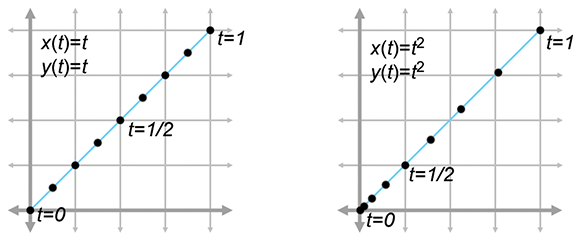Figure 13.1 Two curves that define the same “shape,” but not the same “path”

Notice that both curves in Figure 13.1 define the same static shape, but different paths. On the left, the particle moves with constant velocity, but on the right it starts out slowly and accelerates to the finish.

If we are using a curve as a shape and not a path, then this reparameterization doesn't have a visible effect. But that doesn't mean that the derivatives of the curve are irrelevant in the context of shape design. Imagine that we are creating a font using a curve to define a segment of the letter S. In this instance, we might not care about the velocity at any point, but we would care very much about the tangent of the line at any given point. The tangent at a point is the direction the curve is moving at that point, the line that just touches the curve. The tangent is basically the normalized velocity of the curve. Let's formally define the tangent of a curve to be the unit vector pointing in the same direction as the velocity:

The tangent vector
$\mathbf{t}\left(t\right)=\stackrel{^}{\mathbf{v}}\left(t\right)=\frac{\mathbf{v}\left(t\right)}{\parallel \mathbf{v}\left(t\right)\parallel }.$

Higher derivatives also have geometric meaning. The second derivative is related to curvature, which is sometimes denoted $\kappa$ , the lowercase Greek letter kappa. We can define a measure of curvature by considering a circle of a given radius. A circle with radius $r$ has curvature equal to $\kappa =1/r$ everywhere on the circle. A straight portion of a curve has zero curvature, which can be interpreted as the curvature of a circle with infinite radius. The curvature is computed by the formula

Curvature
$\kappa \left(t\right)=\frac{\parallel \mathbf{v}\left(t\right)×\mathbf{a}\left(t\right)\parallel }{{\parallel \mathbf{v}\left(t\right)\parallel }^{3}}.$

# 13.2Polynomial Interpolation

You are probably already familiar with linear interpolation. Given two “endpoint” values, create a function that transitions at a constant rate (spatially, in a straight line) from one to the other. We say that the function interpolates the two control points, meaning that it passes through the control points and can be used to compute intermediate values.

Polynomial interpolation is similar. Given a series of control points, our goal is to construct a polynomial that interpolates them. The degree of the polynomial depends on the number of control points. A polynomial of degree $n$ can be made to interpolate $n+1$ control points. For example, linear interpolation is simply first-degree polynomial interpolation. We're primarily interested in cubic (third-degree) curves in this chapter, so we are creating polynomials that interpolate four control points.

In the context of curve design, to say that a curve interpolates control points is to place specific emphasis on the fact that the curve passes through the control points. This is to be contrasted with a curve that merely approximates the control points, meaning it doesn't pass through thepoints but is attracted to them in some way. We use the word“knot” to refer to control points that are interpolated, invoking the meta-phor of a rope with knots in it. It would seem at first glance that the availability of an interpolation scheme would make any approximation schemeobsolete, but we'll see that approximation techniques do have their advantages.

Polynomial interpolation is a classic problem with several well-studied solutions. Since this is a book on 3D math we cast the discussion primarily in geometric terms, but be aware that most of the literature on polynomial interpolation adopts a more general view, because the task of fitting a function to a set of data points has broad applicability.

To facilitate the discussion we use a particular example curve, shown in Figure 13.2. It's somewhat like an S turned on its side. We've marked the four control points on the curve that we are attempting to interpolate. We've chosen to place the $y$ coordinates on the interval $\left[2,3\right]$ for reasons that will be useful later.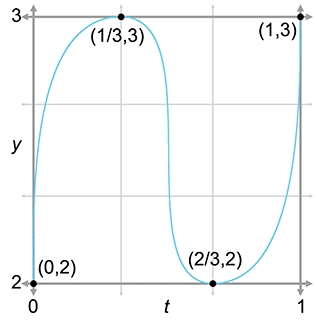$\begin{array}{ccc}t& x\left(t\right)& y\left(t\right)\\ 0& 0& 2\\ 1/3& 1/3& 3\\ 2/3& 2/3& 2\\ 1& 1& 3\end{array}$
Figure 13.2An example curve and four control points. Can we draw this shape?

Notice that we must specify not only the position of each control point (the $x$ and $y$ coordinates), but the time when we want the curve to reach that control point (the $t$ value). We use the notation that the independent value (the “time values”) of the control points are named ${t}_{1},{t}_{2},\dots ,{t}_{n}$ and the dependent variables (the spatial coordinate values at those times) are ${y}_{1},{y}_{2},\dots ,{y}_{n}.$ The symbol $P$ stands for the polynomial function that we seek: ${y}_{i}=P\left({t}_{i}\right)$ .

The array of time values ${t}_{1}\dots {t}_{n}$ is known in other contexts as the knot vector or knot sequence. The word “vector” indicates that the sequence of $t$ values is an array of numbers, not that these numbers form a vector in the geometric sense of the word. If the $t$ s are spaced evenly like they are in our example, then we have a uniform knot vector; otherwise, we say that the knot vector is nonuniform. (Because it might be confusing, let us clarify that the knot vector is the sequence of $t$ values, not the sequence of control points.)

What about the $x$ -coordinate? Because the $x$ and $y$ coordinates are independent of one another, a general 2D curve-fitting application involves two separate one-dimensional problems. Aside from the fact that the two problems use the same knot vector, the coordinates are otherwise unrelated. Even though Figure 13.2 may look like a 2D curve, it is more properly interpreted as a graph of one coordinate (the $y$ -coordinate) as a function of time. We chose as the example curve an S turned on its side, rather than an S in its regular orientation, since the latter is not the graph of a function (technically it's called a relation because it associates more than one value of $y$ with each value of $x$ ).

With that said, there are two ways of interpreting Figure 13.2. We can interpret it either as a 1D function of $y\left(t\right)$ , or as a 2D curve, where one of the coordinates has a trivial form $x=t$ . This is a common source of confusion when looking at diagrams of curves in this book and elsewhere. Make sure you pay special attention to the horizontal axis to make sure you know whether it is a graph of one coordinate over time or a plot of the 2D curve that includes the behavior of both spatial coordinates. The traditional literature on polynomial interpolation is mostly in abstract terms of any function of the form $y=f\left(x\right)$ . In this context, $x$ would be the independent variable rather than a dependent value as it is for us. The notation we have chosen avoids the symbol $x$ and its associated baggage.

Now we are ready to answer a question some readers might be thinking: “I don't care what time the curve reaches the points, I just want a smooth shape that goes through the points.” Unfortunately, this doesn't unambiguously define a curve—we need to provide some other criteria to nail down the shape, and one way to do this is to associate time values with each control point. In typical applications of polynomial interpolation, we want to be able to specify the values of the dependent variable, because we are trying to fit a function to some known data points. There are some reasonable ways we can synthesize this information if we don't have it—for example, by making the difference between adjacent $t$ values proportional to the Euclidian distance between the corresponding control points. However, the general fact that polynomial interpolation needs us to provide the $t$ values when we often don't have a good way to decide what they should be is a harbinger of later discoveries.

Now that we've set the ground rules, let's try to create this curve. We first take a geometric approach in Section 13.2.1. Then, in Section 13.2.2, we look at the problem from a slightly more abstract mathematical perspective.

## 13.2.1Aitken's Algorithm

Our first approach to polynomial interpolation is a recursive technique due to Alexander Aitken (1895–1967). Like many recursive algorithms, it works on the principle of divide and conquer. To solve a difficult problem, we first divide it into two (or more) easier problems, solve the easier problems independently, and then combine the results to get the solution to the harder problem. In this case, the “hard” problem is to create a curve that interpolates $n$ control points. We split this curve into two “easier” curves: (1) one that interpolates only the first $n-1$ points, disregarding the last point; and (2) another that interpolates the last $n-1$ points without worrying about the first point. Then, we blend these two curves together.

Let's take the important cubic (third-degree) case as an example. A cubic curve has four control points ${y}_{1}\dots {y}_{4}$ that we wish to interpolate at the corresponding times ${t}_{1}\dots {t}_{4}$ . Applying the “divide-and-conquer” approach, we split this up into two smaller problems: one curve to interpolate ${y}_{1}\dots {y}_{3}$ , and another curve to interpolate ${y}_{2}\dots {y}_{4}$ . Since each of these curves has three control points, they are quadratic (second-degree) curves. Of course, quadratic curve-fitting is still a “hard” problem for us, and so each curve must be further subdivided.

Consider the first quadratic curve, between ${y}_{1}$ , ${y}_{2}$ , and ${y}_{3}$ . We further divide this curve into two parts, the first part between ${y}_{1}$ and ${y}_{2}$ and the other part between ${y}_{2}$ and ${y}_{3}$ . These two curves have only two control points each; they are straight line segments. Finally, a problem that is truly “easy”!

Since we have lots of curves at this point, we should invent some notation for them. We let ${y}_{i}^{1}\left(t\right)$ denote the linear curve between ${y}_{i}$ and ${y}_{i+1}$ , the notation ${y}_{i}^{2}\left(t\right)$ denote the quadratic curve between ${y}_{i}$ and ${y}_{i+2}$ , and so on. In other words, the superscript indicates the recursion level in the divide-and-conquer algorithm (and also the degree of the polynomial), and the subscript indexes along the length of the curve.

Take a look at the first quadratic curve ${y}_{1}^{2}\left(t\right)$ that interpolates ${y}_{1}$ , ${y}_{2}$ , and ${y}_{3}$ . It is formed by blending together the two lines containing the first two linear segments. An example of such blending is shown in Figure 13.3. (This figure doesn't use the data from our S example; it's a less symmetric case that better illustrates the blending process.) Notice that each curve segment is an interval from an infinite curve that is defined for any value of $t$ .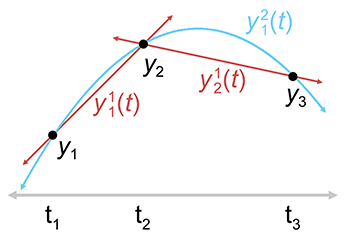Figure 13.3 Creating a quadratic curve as a blend of two linear segments according to Aitken's algorithm

Now let's look at the math behind this. It's all linear interpolation. The easiest are the linear segments, which are defined by linear interpolation between the adjacent control points:

Linear interpolation between two control points
$\begin{array}{rlrl}{y}_{1}^{1}\left(t\right)& =\frac{\left({t}_{2}-t\right){y}_{1}+\left(t-{t}_{1}\right){y}_{2}}{{t}_{2}-{t}_{1}},& {y}_{2}^{1}\left(t\right)& =\frac{\left({t}_{3}-t\right){y}_{2}+\left(t-{t}_{2}\right){y}_{3}}{{t}_{3}-{t}_{2}}.\end{array}$

The quadratic curve is only slightly more complicated. We just linearly interpolate between the line segments:

Linear interpolation of lines yields a quadratic curve
${y}_{1}^{2}\left(t\right)=\frac{\left({t}_{3}-t\right)\left[{y}_{1}^{1}\left(t\right)\right]+\left(t-{t}_{1}\right)\left[{y}_{2}^{1}\left(t\right)\right]}{{t}_{3}-{t}_{1}}.$

Hopefully you can see the pattern—each curve is the result of linearly interpolating two curves of lesser degree. Aitken's algorithm can be summarized succinctly as a recurrence relation.

Aitken's Algorithm
$\begin{array}{rl}{y}_{i}^{0}\left(t\right)& ={y}_{i},\\ {y}_{i}^{j}\left(t\right)& =\frac{\left({t}_{i+j}-t\right)\left[{y}_{i}^{j-1}\left(t\right)\right]+\left(t-{t}_{i}\right)\left[{y}_{i+1}^{j-1}\left(t\right)\right]}{{t}_{i+j}-{t}_{i}}.\end{array}$

Aitken's algorithm works because, at each level both curves being blended already touch the middle control points. The two outermost control points are touched by only one curve or the other, but for those values of $t$ , the blend weights reach their extreme values and all the weight is given to the curve that touches the control point.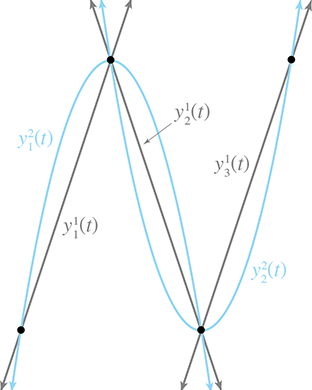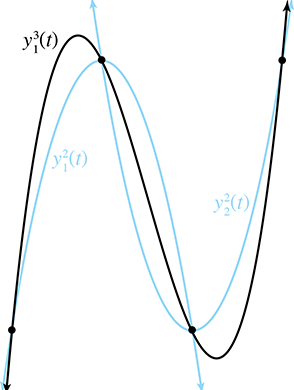Figure 13.4Two levels of Aitken's algorithm

Now that we have the basic idea, let's apply it to our sideways S. Figure 13.4 shows Aitken's algorithm at work with our four data points. On the left, the three linear segments are blended to form two quadratic segments. On the right, the two quadratic curves are blending, yielding the final result that we've been seeking: a cubic spline that interpolates all four control points.

So we've successfully interpolated the four control points, and accomplished the goal set out at the start of this section, right? Well, not exactly. Although our curve does pass through the control points, it isn't really the curve we wanted. If we compare the curve on the right side of Figure 13.4 with the curve we set out to create at the start of this section in Figure 13.2, we see that the curve produced by Aitken's algorithm overshoots the $y$ value of the two middle control points. We have discovered an inconvenient truth.2

Polynomial interpolation doesn't really give us the type of control we want for curve design in geometric settings.

But don't despair! We've learned several important ideas that will be helpful when we discuss Bézier curves in Section 13.4 and splines in Section 13.6. In fact, we're going to beg your patience to allow us to extend the discussion on polynomial interpolation just a bit further. It's sort of like watching the movie Titanic; even though you know that the journey will end tragically, you still might find something useful along the way. We promise that the other techniques in this chapter will have practical as well as educational value.

By the way, you might have noticed that we didn't actually compute the polynomial $P$ that produces the curve. Working through this math is straightforward, but a bit tedious and not all that enlightening. The important point is that Aitken's algorithm is a recursive process of blending curves together and works by repeated linear interpolation. Besides, why bother with the details when we have computers to solve algebra problems for us?3 However, you needn't feel short-changed by lazy authors. If you really want to know what the polynomial is (or just want to feel like you're getting your money's worth), keep reading. We'll discover it in the next section by using a different method that's less tedious mathematically.

## 13.2.2Lagrange Basis Polynomials

Section 13.2.1 applied geometric intuition to the problem of polynomial interpolation and came up with Aitken's algorithm. Now we approach the subject from a more abstract mathematical point of view.

One mathematical approach to the interpolation problem comes from linear algebra.4 Each control point gives us one equation, and each coefficient gives us one unknown. This system of equations can be put into an $n×n$ matrix,5 which can be solved by standard techniques such as Gaussian elimination or LU decomposition. Such techniques are outside the scope of this book, but you can learn about them in practically any good book on linear algebra or numerical methods.

Solving a matrix is a relatively time-consuming computational process, requiring $O\left({n}^{3}\right)$ time for an $n×n$ matrix in the worst case. Luckily there are more efficient approaches. As we did with Aitken's algorithm, we solve a large complicated problem by dividing it into a series of smaller, simpler problems, and then combining those results. Aitken's algorithm is a recursive procedure, but here we will make one “simple” problem per control point.

Let's ignore the $y$ 's for now and think only about the $t$ 's. What if we could create a polynomial for each knot ${t}_{i}$ such that the polynomial evaluates to unity at that knot, but for all the other knots it evaluates to zero? If we denote the $i$ th polynomial as ${\ell }_{i}$ , then this idea can be expressed in mathspeak: ${\ell }_{i}\left({t}_{i}\right)=1$ , and ${\ell }_{i}\left({t}_{j}\right)=0$ for all $j\ne i$ . For example, let's assume $n=4$ . Then our polynomials would have the following values at the knots:

$\begin{array}{rlrlrlrl}{\ell }_{1}\left({t}_{1}\right)& =1,& {\ell }_{1}\left({t}_{1}\right)& =0,& {\ell }_{3}\left({t}_{1}\right)& =0,& {\ell }_{4}\left({t}_{1}\right)& =0,\\ {\ell }_{1}\left({t}_{2}\right)& =0,& {\ell }_{2}\left({t}_{2}\right)& =1,& {\ell }_{3}\left({t}_{2}\right)& =0,& {\ell }_{4}\left({t}_{2}\right)& =0,\\ {\ell }_{1}\left({t}_{3}\right)& =0,& {\ell }_{2}\left({t}_{3}\right)& =0,& {\ell }_{3}\left({t}_{3}\right)& =1,& {\ell }_{4}\left({t}_{3}\right)& =0,\\ {\ell }_{1}\left({t}_{4}\right)& =0,& {\ell }_{2}\left({t}_{4}\right)& =0,& {\ell }_{3}\left({t}_{4}\right)& =0,& {\ell }_{4}\left({t}_{4}\right)& =1.\end{array}$

If we were able to create polynomials with the above properties, we would be able to use them as basis polynomials. We would scale each basis polynomial ${\ell }_{i}$ by the corresponding coordinate value ${y}_{i}$ , and add all the scaled polynomials together:

Interpolating polynomial in Lagrange basis form
$\begin{array}{}\text{(13.7)}& P\left(t\right)=\sum _{i=1}^{n}{y}_{i}{\ell }_{i}\left(t\right)={y}_{1}{\ell }_{1}\left(t\right)+{y}_{2}{\ell }_{2}\left(t\right)+\cdots +{y}_{n-1}{\ell }_{n-1}\left(t\right)+{y}_{n}{\ell }_{n}\left(t\right).\end{array}$

You might want to take a moment to convince yourself that this polynomial actually interpolates the control points, meaning $P\left({t}_{i}\right)={y}_{i}$ .

Notice that the basis polynomials depend only on the knot vector (the $t$ 's) and not on the coordinate values (the $y$ 's). Because of this, a set of basis polynomials can be used to quickly construct multiple curves with the same knot vector. This is precisely the situation we find ourselves in when dealing with a 3D curve, which is really three one-dimensional curves that share the same knot sequence.

Of course, all of this would work only if we knew the basis polynomials, and finding ${\ell }_{i}$ is itself a problem of polynomial interpolation. However, the “data points” we wish ${\ell }_{i}$ to interpolate are all either 0 or 1, so ${\ell }_{i}$ can be expressed in a simple form. Such basis polynomials are called Lagrange basis polynomials.6 A Lagrange7 basis polynomial ${\ell }_{i}$ for knot vector ${t}_{1}\dots {t}_{n}$ looks like Equation (13.8):

Lagrange Basis Polynomial

This trick works because at the knot ${t}_{i}$ , all the terms in the product equal 1, causing the entire expression to evaluate to 1, and at any other knot, one of the terms in the product is 0, which causes the entire expression to evaluate to 0.

Let's apply this to our example S curve. Recall that it used the uniform knot vector $\left(0,\frac{1}{3},\frac{2}{3},1\right)$ . Here, we work through the first basis polynomial and just present the results for the others:

$\begin{array}{rl}{\ell }_{1}\left(t\right)& =\left(\frac{t-{t}_{2}}{{t}_{1}-{t}_{2}}\right)\left(\frac{t-{t}_{3}}{{t}_{1}-{t}_{3}}\right)\left(\frac{t-{t}_{4}}{{t}_{1}-{t}_{4}}\right)=\left(\frac{t-1/3}{0-1/3}\right)\left(\frac{t-2/3}{0-2/3}\right)\left(\frac{t-1}{0-1}\right)\\ & =\left(\frac{3t-1}{-1}\right)\left(\frac{3t-2}{-2}\right)\left(\frac{t-1}{-1}\right)=\frac{\left(3t-1\right)\left(3t-2\right)\left(t-1\right)}{-2}\\ & =-\left(9/2\right){t}^{3}+9{t}^{2}-\left(11/2\right)t+1,\end{array}$
$\begin{array}{rl}{\ell }_{2}\left(t\right)& =\left(27/2\right){t}^{3}-\left(45/2\right){t}^{2}+9t,\\ {\ell }_{3}\left(t\right)& =-\left(27/2\right){t}^{3}+18{t}^{2}-\left(9/2\right)t,\\ {\ell }_{4}\left(t\right)& =\left(9/2\right){t}^{3}-\left(9/2\right){t}^{2}+t.\end{array}$

Figure 13.5 shows what these basis polynomials look like.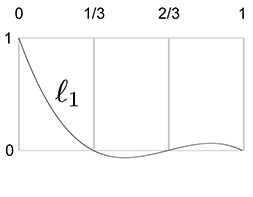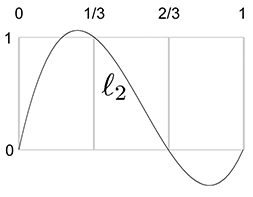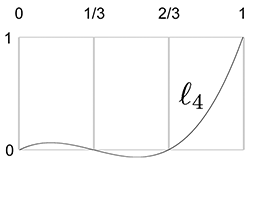Figure 13.5Cubic Lagrange basis polynomials for uniform knot vector

Now that we have the Lagrange basis polynomials for the knot vector, let's plug in the $y$ values from our example S curve (Figure 13.2) into Equation (13.7) to get the complete interpolating polynomial:

$\begin{array}{rl}P\left(t\right)& ={y}_{1}{\ell }_{1}\left(t\right)+{y}_{2}{\ell }_{2}\left(t\right)+{y}_{3}{\ell }_{3}\left(t\right)+{y}_{4}{\ell }_{4}\left(t\right)\\ & =2\left[-\left(9/2\right){t}^{3}+9{t}^{2}-\left(11/2\right)t+1\right]+3\left[\left(27/2\right){t}^{3}-\left(45/2\right){t}^{2}+9t\right]\\ & \phantom{\rule{1em}{0ex}}\phantom{\rule{1em}{0ex}}+2\left[-\left(27/2\right){t}^{3}+18{t}^{2}-\left(9/2\right)t\right]+3\left[\left(9/2\right){t}^{3}-\left(9/2\right){t}^{2}+t\right]\\ & =-9{t}^{3}+18{t}^{2}-11t+2+\left(81/2\right){t}^{3}-\left(135/2\right){t}^{2}+27t\\ & \phantom{\rule{1em}{0ex}}\phantom{\rule{1em}{0ex}}-27{t}^{3}+36{t}^{2}-9t+\left(27/2\right){t}^{3}-\left(27/2\right){t}^{2}+3t\\ & =18{t}^{3}-27{t}^{2}+10t+2.\end{array}$

Let's show these results graphically. First, we scale each basis polynomial by the corresponding coordinate value, as shown in Figure 13.6.

Finally, adding the scaled basis vectors together yields the interpolating polynomial $P$ , the blue curve at the top of Figure 13.7.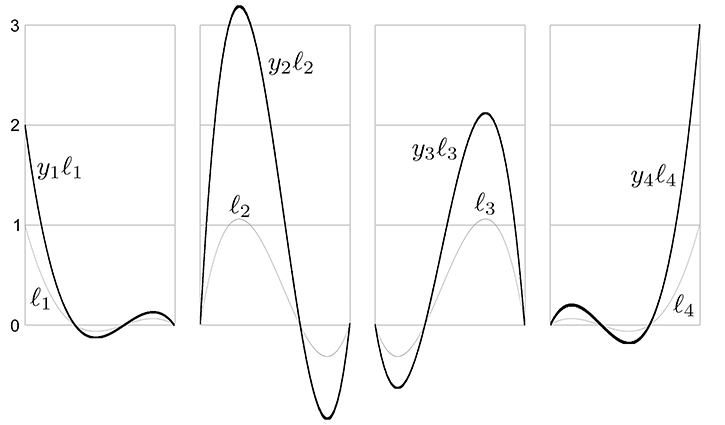Figure 13.6 Scaling each Lagrange basis polynomial by the corresponding coordinate value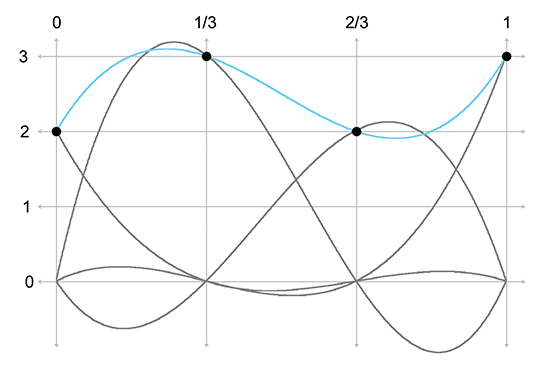Figure 13.7 The interpolating curve is the sum of the scaled basis polynomials

We use the word basis in basis polynomial to emphasize the fact that we can use these polynomials as building blocks to reconstruct absolutely any polynomial whatsoever, given the values of the polynomial at the knots. It's the same basic concept as a basis vector (see Section 3.3.3): any arbitrary vector can be described as a linear combination of the basis vectors. In our case, the space being spanned by the basis is not a geometric 3D space, but the vector space of all possible polynomials of a certain degree, and the scale values for each curve are the known values of the polynomial at the knots.

But there's an alternate way to understand the multiplication and summing that's going on. Instead of thinking about the polynomials as the building blocks and the control points as the scale factors, we can view each point on the curve as a result of taking a weighted average of the control points, where the basis polynomials provide the blending weights. So the control points are the building blocks and the basis polynomials provide the scale factors, although we prefer to be more specific and call these scale factors barycentric coordinates. We introduced barycentric coordinates in the context of triangles in Section 9.6.3, but the term refers to a general technique of describing some value as a weighted average of data points.

We can think of basis polynomials as functions yielding barycentric coordinates (blending weights).

Notice that some values are negative or greater than 1 on certain intervals, which explains why direct polynomial interpolation overshoots the control points. When all barycentric coordinates are inside the $\left[0,1\right]$ range, the resulting point is guaranteed to lie inside the convex hull of the control points. (The convex hull is the smallest polygon that contains all the control points. It “shrink wraps” the control points, sort of like if you were to stretch a rubber band around the control points and then release it.) But when we have any one coordinate outside this interval, the resulting point could extend outside the convex hull. For purposes of geometric curve design, the convex hull guarantee is a very nice one to have. Section 13.4 shows that Bézier curves do provide this guarantee through the Bernstein basis.

## 13.2.3Polynomial Interpolation Summary

We've approached polynomial interpolation from two perspectives. Aitken's algorithm is a geometric approach based on repeated linear interpolation, and with it we can compute a point on the curve for a given $t$ without knowing the polynomial for the curve. Lagrange interpolation works by creating basis functions that depend only on the knot vector. We can view the use of the basis polynomials in two ways. Either we can think about scaling each basis polynomial by the corresponding coordinate value and then adding them all together, or we can think about the polynomials as functions that compute barycentric coordinates that are used as blending weights in a simple weighted average of the coordinate points.

Both methods yield the same curve when given the same data. Furthermore, this polynomial is unique—no other polynomial of the same degree interpolates the data points. An informal argument for why this is true goes like this: A polynomial of degree $n$ has $n+1$ degrees of freedom, corresponding to the $n+1$ coefficients in monomial form. Therefore, the degree $n$ polynomial that interpolates $n+1$ control points must be unique. (Farin  gives a more rigorous argument.)

For purposes of curve design, polynomial interpolation is not ideal, primarily because of our inability to control the overshoot. The overshoot is guaranteed by the fact that the underlying Lagrange basis polynomials are not restricted to the unit interval $\left[0,1\right]$ , and the curve escapes the convex hull of the control points.

Direct polynomial interpolation finds limited application in video games, but our study has introduced the themes of repeated linear interpolation and basis polynomials. We've also seen a bit of the beautiful duality between the two techniques.

# 13.3Hermite Curves

Polynomial interpolation tries to control the interior of the curve by threading the curve through specified knots. This doesn't work as well as we would like, because of the tendency to oscillate and overshoot, so let's try a different approach. We're still going to want to specify the endpoint positions, of course. But instead of specifying the interior positions to interpolate, let's control the shape of the curve through the tangents at the endpoints. A curve thus specified is said to be a Hermite curve or a curve in Hermite form, named in honor of Charles Hermite8 (1822–1901).

The Hermite form specifies a curve by listing its starting and ending positions and derivatives. A cubic curve has only four coefficients, which allows for the specification of just the first derivatives, the velocities at the endpoints. So describing a cubic curve in Hermite form boils down to the following four pieces of information:

• The start position at $t=0$ ,
• The first derivative (initial velocity) at $t=0$ ,
• The end position at $t=1$ ,
• The first derivative (final velocity) at $t=1$ .

Let's call the desired start and end positions ${\mathbf{p}}_{0}$ and ${\mathbf{p}}_{1}$ and the start and end velocities ${\mathbf{v}}_{0}$ and ${\mathbf{v}}_{1}$ . Figure 13.8 shows some examples of cubic Hermite curves. Please note that the velocity vectors ${\mathbf{v}}_{0}$ and ${\mathbf{v}}_{1}$ have been drawn at one-third their actual length. One reason for doing this is to save space, and another will make sense later once we learn about Bézier curves in Section 13.4.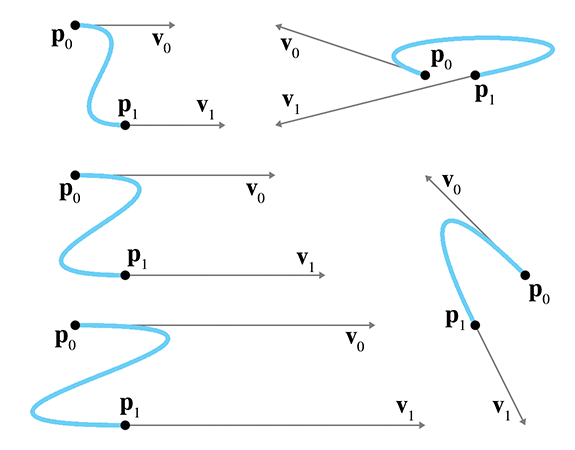Figure 13.8Some cubic Hermite curves

Determining the monomial coefficients from the Hermite values is a relatively straightforward algebraic process of combining equations previously discussed in this chapter. The four Hermite values can be translated into the following system of equations:

$\begin{array}{}\text{(13.9)}& \mathbf{p}\left(0\right)& ={\mathbf{p}}_{0}& ⟹& & {\mathbf{c}}_{0}& ={\mathbf{p}}_{0},& & \text{(13.10)}& \mathbf{v}\left(0\right)& ={\mathbf{v}}_{0}& ⟹& & {\mathbf{c}}_{1}& ={\mathbf{v}}_{0},& & \text{(13.11)}& \mathbf{v}\left(1\right)& ={\mathbf{v}}_{1}& ⟹& & {\mathbf{c}}_{1}+2{\mathbf{c}}_{2}+3{\mathbf{c}}_{3}& ={\mathbf{v}}_{1},& & \text{(13.12)}& \mathbf{p}\left(1\right)& ={\mathbf{p}}_{1}& ⟹& & {\mathbf{c}}_{0}+{\mathbf{c}}_{1}+{\mathbf{c}}_{2}+{\mathbf{c}}_{3}& ={\mathbf{p}}_{1}.& & \end{array}$
System of equations for Hermite conditions

Equations (13.9) and (13.12), which specify the endpoints, just repeat what we said in Section 13.1.5. Equations (13.10) and (13.11), which specify velocities, follow directly from the velocity equations for a cubic polynomial (Equation (13.5). The order in which these equations are listed is a convention used in other literature on curves, and the utility of this convention will become apparent later in this chapter.

Solving this system of equations results in a method to compute the monomial coefficients from the Hermite positions and derivatives:

Converting Hermite form to monomial form
$\begin{array}{rl}\text{(13.13)}& {\mathbf{c}}_{0}& ={\mathbf{p}}_{0},{\mathbf{c}}_{1}& ={\mathbf{v}}_{0},\\ {\mathbf{c}}_{2}& =-3{\mathbf{p}}_{0}-2{\mathbf{v}}_{0}-{\mathbf{v}}_{1}+3{\mathbf{p}}_{1},\\ \text{(13.16)}& {\mathbf{c}}_{3}& =2{\mathbf{p}}_{0}+{\mathbf{v}}_{0}+{\mathbf{v}}_{1}-2{\mathbf{p}}_{1}.\end{array}$

We can also write these equations in the compact matrix notation introduced in Section 13.1.2. Remember that when we put the coefficients as columns in a matrix $\mathbf{C}$ , and the powers of $t$ into the column vector $\mathbf{t}$ , we can express a polynomial curve as the matrix product $\mathbf{C}\mathbf{t}$ ,

We can write monomial form using matrix notation, remember?
$\mathbf{p}\left(t\right)=\mathbf{C}\mathbf{t}=\left[\begin{array}{cccc}|& |& |& |\\ {\mathbf{c}}_{0}& {\mathbf{c}}_{1}& {\mathbf{c}}_{2}& {\mathbf{c}}_{3}\\ |& |& |& |\end{array}\right]\left[\begin{array}{c}1\\ t\\ {t}^{2}\\ {t}^{3}\end{array}\right],$

where $\mathbf{p}\left(t\right)$ and each of the coefficient vectors ${\mathbf{c}}_{i}$ are column vectors whose height matches the number of geometric dimensions (1D, 2D, or 3D). The height of $\mathbf{t}$ matches the number of $\mathbf{c}$ 's, which depends on the degree of the curve.

The coefficient matrix $\mathbf{C}$ may be expressed as a matrix product by putting the Hermite positions and velocities as columns in a matrix $\mathbf{P}$ and multiplying by a conversion matrix $\mathbf{H}$ :

Cubic Hermite curve using matrix notation
$\mathbf{p}\left(t\right)=\mathbf{C}\mathbf{t}=\mathbf{P}\mathbf{H}\mathbf{t}=\left[\begin{array}{cccc}|& |& |& |\\ {\mathbf{p}}_{0}& {\mathbf{v}}_{0}& {\mathbf{v}}_{1}& {\mathbf{p}}_{1}\\ |& |& |& |\end{array}\right]\left[\begin{array}{cccc}1& 0& -3& 2\\ 0& 1& -2& 1\\ 0& 0& -1& 1\\ 0& 0& 3& -2\end{array}\right]\left[\begin{array}{c}1\\ t\\ {t}^{2}\\ {t}^{3}\end{array}\right].$

We can interpret the product $\mathbf{P}\mathbf{H}\mathbf{t}$ in two ways. If we group it like $\mathbf{P}\left(\mathbf{H}\mathbf{t}\right)$ , then the matrix product $\mathbf{H}\mathbf{t}$ can be interpreted as Hermite basis functions; we'll have more to say about this basis shortly. Or, we can think about $\mathbf{C}=\mathbf{P}\mathbf{H}$ , in which case, multiplication by $\mathbf{H}$ can be considered a conversion from the Hermite basis to the monomial basis, essentially a restatement of Equations (13.13)(13.16).

We emphasize that the adjectives “monomial,” “Hermite,” and “Bézier” refer to different ways of describing the same set of polynomial curves; they are not different sets of curves. We convert a curve from Hermite form to monomial form by using Equations (13.13)(13.16), and from monomial form to Hermite form with Equations (13.9)(13.12).

Let's take a closer look at the Hermite basis and hopefully gain some geometric intuition as to why it works. Remember that we can interpret basis functions as functions of $t$ yielding barycentric coordinates. For cubic Hermite curves, four values are being blended: the two positions and the two velocity vectors.9 Thus, we have four basis functions that are the elements of the column result of the matrix product $\mathbf{H}\mathbf{t}$ . Expanding the product, we have

$\begin{array}{rl}\mathbf{p}\left(t\right)& =\mathbf{P}\left(\mathbf{H}\mathbf{t}\right)\\ & =\left[\begin{array}{cccc}|& |& |& |\\ {\mathbf{p}}_{0}& {\mathbf{v}}_{0}& {\mathbf{v}}_{1}& {\mathbf{p}}_{1}\\ |& |& |& |\end{array}\right]\left(\left[\begin{array}{cccc}1& 0& -3& 2\\ 0& 1& -2& 1\\ 0& 0& -1& 1\\ 0& 0& 3& -2\end{array}\right]\left[\begin{array}{c}1\\ t\\ {t}^{2}\\ {t}^{3}\end{array}\right]\right)\\ & =\left[\begin{array}{cccc}|& |& |& |\\ {\mathbf{p}}_{0}& {\mathbf{v}}_{0}& {\mathbf{v}}_{1}& {\mathbf{p}}_{1}\\ |& |& |& |\end{array}\right]\left[\begin{array}{c}1-3{t}^{2}+2{t}^{3}\\ t-2{t}^{2}+{t}^{3}\\ -{t}^{2}+{t}^{3}\\ 3{t}^{2}-2{t}^{3}\end{array}\right].\end{array}$

Next, we name these basis functions (the rows of $\mathbf{H}\mathbf{t}$ ) as ${H}_{0}\phantom{\rule{-0.2ex}{0ex}}\left(t\right)\dots {H}_{3}\phantom{\rule{-0.2ex}{0ex}}\left(t\right)$ (you may see these same functions indexed with different subscripts in other sources):

The cubic Hermite basis functions
$\begin{array}{rl}{H}_{0}\phantom{\rule{-0.2ex}{0ex}}\left(t\right)& =1-3{t}^{2}+2{t}^{3},\\ {H}_{1}\phantom{\rule{-0.2ex}{0ex}}\left(t\right)& =t-2{t}^{2}+{t}^{3},\\ {H}_{2}\phantom{\rule{-0.2ex}{0ex}}\left(t\right)& =-{t}^{2}+{t}^{3},\\ {H}_{3}\phantom{\rule{-0.2ex}{0ex}}\left(t\right)& =3{t}^{2}-2{t}^{3}.\end{array}$

Now, expanding the matrix multiplication makes it explicit that these functions serve as blending weights:

Interpreting the Hermite basis functions as blending weights
$\begin{array}{rl}\mathbf{p}\left(t\right)& =\left[\begin{array}{cccc}|& |& |& |\\ {\mathbf{p}}_{0}& {\mathbf{v}}_{0}& {\mathbf{v}}_{1}& {\mathbf{p}}_{1}\\ |& |& |& |\end{array}\right]\left[\begin{array}{c}{H}_{0}\phantom{\rule{-0.2ex}{0ex}}\left(t\right)\\ {H}_{1}\phantom{\rule{-0.2ex}{0ex}}\left(t\right)\\ {H}_{2}\phantom{\rule{-0.2ex}{0ex}}\left(t\right)\\ {H}_{3}\phantom{\rule{-0.2ex}{0ex}}\left(t\right)\end{array}\right]\\ & ={H}_{0}\phantom{\rule{-0.2ex}{0ex}}\left(t\right)\phantom{\rule{thinmathspace}{0ex}}{\mathbf{p}}_{0}+{H}_{1}\phantom{\rule{-0.2ex}{0ex}}\left(t\right)\phantom{\rule{thinmathspace}{0ex}}{\mathbf{v}}_{0}+{H}_{2}\phantom{\rule{-0.2ex}{0ex}}\left(t\right)\phantom{\rule{thinmathspace}{0ex}}{\mathbf{v}}_{1}+{H}_{3}\phantom{\rule{-0.2ex}{0ex}}\left(t\right)\phantom{\rule{thinmathspace}{0ex}}{\mathbf{p}}_{1}.\end{array}$

Figure 13.9 shows a graph of the Hermite basis functions.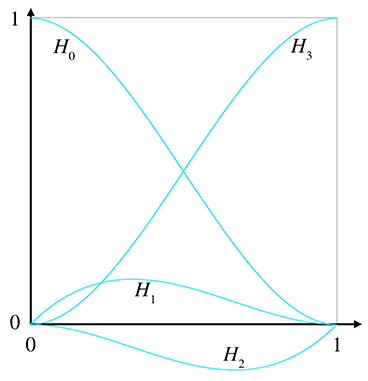Figure 13.9The Hermite basis functions

Now let's make a few observations. First, notice that ${H}_{0}\phantom{\rule{-0.2ex}{0ex}}\left(t\right)+{H}_{3}\phantom{\rule{-0.2ex}{0ex}}\left(t\right)=1$ , so those who object to the idea of adding “points” together can breath a sigh of relief, as we can interpret the situation as a proper barycentric combination of the points.

The curve ${H}_{3}\phantom{\rule{-0.2ex}{0ex}}\left(t\right)$ deserves special attention. It is also is known as the smoothstep function and is truly a gem that every game programmer should know. This function is found in many places, including the Renderman shading language and HLSL. To remove the rigid, robotic feeling from any linear interpolation (especially camera transitions), simply compute the normalized interpolation fraction $t$ as usual (in the range $0\le t\le 1$ ), and then replace $t$ with $3{t}^{2}-2{t}^{3}$ . Voila! Everything will suddenly feel more polished. The reason for this is that the smoothstep function eliminates the sudden jump in velocity at the endpoints: ${H}_{3}^{\prime }\left(0\right)={H}_{3}^{\prime }\left(1\right)=0$ .

The Hermite basis function ${H}_{3}\phantom{\rule{-0.2ex}{0ex}}\left(t\right)$ is also known as the smoothstep function. Almost any transition based on linear interpolation, especially a camera transition, feels better when replaced with the smoothstep function.

One final word about Hermite curves. Like the other forms for polynomial curves, it's possible to design a scheme for Hermite curves of higher degree, although the cubic polynomial is the most commonly used in computer graphics and animation. With the cubic spline, we specified the position (the “0th” derivative) and velocities (first derivatives) at the end points. A quintic (fifth-degree) Hermite curve happens when we also specify the accelerations (second derivatives).

# 13.4Bézier Curves

This chapter has so far discussed a number of ideas about curves that were enlightening, but it has yet to describe a fully practical way to design a curve. All of that will change in this section.10 Bézier curves were invented by Pierre Bézier (1910–1999), a French11 engineer, while he was working for the automaker Renault. Bézier curves have many desirable properties that make them well suited for curve design. Importantly, Bézier curves approximate rather than interpolate: although they do pass through the first and last control points, they only pass near the interior points. For this reason, the Bézier control points are called “control points” rather than “knots.” Some example cubic Bézier curves are shown in Figure 13.10.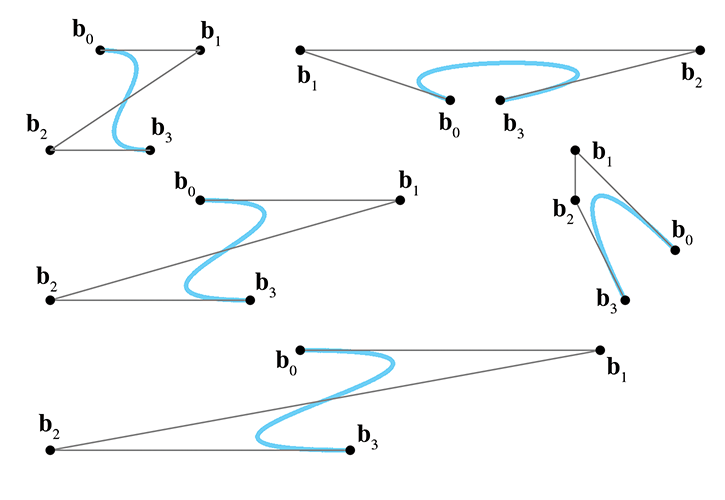Figure 13.10Some cubic Bézier curves

Recall from Section 13.2 that the problem of polynomial interpolation had two solutions that produced the same result. Aitken's algorithm was a recursive construction technique that appealed to our geometric sensibilities, and a more abstract approach yielded the Lagrange basis polynomials. Bézier curves exhibit a similar duality. The counterpart of Aitken's algorithm for Bézier curves is the de Casteljau algorithm, a recursive geometric technique for constructing Bézier curves through repeated linear interpolation; this is the subject of Section 13.4.1. The analog to the Lagrange basis is the Bernstein basis, which is discussed in Section 13.4.2. After considering both sides of this coin, Section 13.4.3 investigates the derivatives12 of Bézier curves and reveals the relationship to Hermite form.

We've seen some beautiful cooperation between math and geometry in this book, but the convergence is particularly elegant for Bézier curves. It seems as if almost every important property of Bézier curves was independently discovered multiple times by researchers in different fields. Rogers' book  includes an interesting look at this story.

 $t=.25$ $t=.50$ $t=.75$ Unknown environment 'picture' $\text{Unknown environment 'picture'}$ Unknown environment 'picture' $\text{Unknown environment 'picture'}$ Unknown environment 'picture' $\text{Unknown environment 'picture'}$ Unknown environment 'picture' $\text{Unknown environment 'picture'}$ Unknown environment 'picture' $\text{Unknown environment 'picture'}$ Unknown environment 'picture' $\text{Unknown environment 'picture'}$ Unknown environment 'picture' $\text{Unknown environment 'picture'}$ Unknown environment 'picture' $\text{Unknown environment 'picture'}$ Unknown environment 'picture' $\text{Unknown environment 'picture'}$ Unknown environment 'picture' $\text{Unknown environment 'picture'}$ Unknown environment 'picture' $\text{Unknown environment 'picture'}$ Unknown environment 'picture' $\text{Unknown environment 'picture'}$
Figure 13.11The de Casteljau algorithm applied to a cubic curve

## 13.4.1The de Casteljau Algorithm

The de Casteljau algorithm defines a method for constructing Bézier curves through repeated linear interpolation. It was created in 1959 by physicist and mathematician Paul de Casteljau (1910–1999).13 We show how the algorithm works for the important cubic case as our example. First, a bit of notation is necessary. A cubic curve is defined by four control points, ${\mathbf{b}}_{0}\dots {\mathbf{b}}_{3}$ . Notice that Bézier control points traditionally are indexed starting at zero (which will appeal to the C programmers amongst us). Also, as with Aitken's algorithm, we add a superscript to indicate the level of recursion. The original control points are assigned level 0, thus ${\mathbf{b}}_{i}^{0}={\mathbf{b}}_{i}$ .

With that out of the way, let's consider a specific parameter value $t$ from 0 to 1. The de Casteljau algorithm geometrically constructs the corresponding point on the curve $\mathbf{p}\left(t\right)$ as follows. Between each pair of consecutive control points, we interpolate according to the fraction $t$ to obtain a new point. So, starting with the original four control points ${\mathbf{b}}_{0}^{0}\dots {\mathbf{b}}_{3}^{0}$ , we derive three new points ${\mathbf{b}}_{0}^{1}$ , ${\mathbf{b}}_{1}^{1}$ , and ${\mathbf{b}}_{2}^{1}$ . Another round of interpolation between each pair of these three points gives us two points ${\mathbf{b}}_{0}^{2}$ and ${\mathbf{b}}_{1}^{2}$ , and a final interpolation yields the point ${\mathbf{b}}_{0}^{3}=\mathbf{p}\left(t\right)$ we're looking for. Figure 13.11 shows the de Casteljau algorithm applied to the same curve at $t=.25$ , $t=.50$ , and $t=.75$ .

It's helpful to write out all the $\mathbf{b}$ s in a triangular fashion, as shown in Figure 13.12. Each intermediate point is the result of linearly interpolating between two points on the row above.

$\begin{array}{ccccccccccccc}{\mathbf{b}}_{0}^{0}& & & & {\mathbf{b}}_{1}^{0}& & & & {\mathbf{b}}_{2}^{0}& & & & {\mathbf{b}}_{3}^{0}\\ & ↘& & ↙& & ↘& & ↙& & ↘& & ↙& \\ & & {\mathbf{b}}_{0}^{1}& & & & {\mathbf{b}}_{1}^{1}& & & & {\mathbf{b}}_{2}^{1}& & \\ & & & ↘& & ↙& & ↘& & ↙& & & \\ & & & & {\mathbf{b}}_{0}^{2}& & & & {\mathbf{b}}_{1}^{2}& & & & \\ & & & & & ↘& & ↙& & & & & \\ & & & & & & {\mathbf{b}}_{0}^{3}& & & & & & \end{array}$
Figure 13.12 Hierarchical relationships in the de Casteljau algorithm for a cubic curve

If we combine these recursive relationships with the basic linear interpolation formula, we obtain the de Casteljau recurrence relation.

De Casteljau Recurrence Relation
$\begin{array}{rl}{\mathbf{b}}_{i}^{0}\left(t\right)& ={\mathbf{b}}_{i},\\ {\mathbf{b}}_{i}^{n}\left(t\right)& =\left(1-t\right)\left[{\mathbf{b}}_{i}^{n-1}\phantom{\rule{negativethinmathspace}{0ex}}\left(t\right)\right]+t\left[{\mathbf{b}}_{i+1}^{n-1}\phantom{\rule{negativethinmathspace}{0ex}}\left(t\right)\right].\end{array}$

Listing 13.1 illustrates how the de Casteljau algorithm could be implemented in C++ to evaluate a Bézier curve for a specific value of $t$ . The caller passes in the original control points in an array, which is also used as a temporary working space as the operation is performed in place. Each iteration of the outer loop performs one round of interpolation, replacing the points at one level with the points at the next higher numbered superscript. This process is continued until there is one point remaining, the desired result $\mathbf{p}\left(t\right)$ . This example is intended to illustrate how the algorithm works, not how to do anything particularly fast or provide a clean interface.

Vector3 deCasteljau(
int n,            // order of the curve, the number of points
Vector3 points[], // array of points.  Overwritten, as
// the algorithm works in place
float t           // parameter value we wish to evaluate
) {

// Perform the conversion in place
while (n > 1) {
--n;

// Perform the next round of interpolation, reducing the
// degree of the curve by one.
for (int i = 0 ; i < n ; ++i) {
points[i] = points[i]*(1.0f-t) + points[i+1]*t;
}
}

// Result is now in the first slot.
return points;
}


This gives us a method for locating a point at any given $t$ through repeated interpolation, but it doesn't directly give us a closed form expression to calculate the point in terms of the control points. We emphasize that such a closed form expression is often not needed, but let's derive it in monomial form anyway. We're looking for a polynomial grouped by powers of $t$ . We'll work our way up from the linear and quadratic cases to the cubic. Section 13.4.2 presents a general pattern leading us to the expression for arbitrary degree curves.

The linear case comes straight from the recurrence relation without any real work:

$\begin{array}{rl}{\mathbf{b}}_{i}^{0}\left(t\right)& ={\mathbf{b}}_{i},\\ {\mathbf{b}}_{i}^{1}\left(t\right)& =\left(1-t\right)\left[{\mathbf{b}}_{i}^{0}\phantom{\rule{negativethinmathspace}{0ex}}\left(t\right)\right]+t\left[{\mathbf{b}}_{i+1}^{0}\phantom{\rule{negativethinmathspace}{0ex}}\left(t\right)\right]\\ & =\left(1-t\right){\mathbf{b}}_{i}+t{\mathbf{b}}_{i+1}\\ & ={\mathbf{b}}_{i}+t\left({\mathbf{b}}_{i+1}-{\mathbf{b}}_{i}\right).\end{array}$

Applying one more level gives us a quadratic polynomial:

$\begin{array}{rl}{\mathbf{b}}_{i}^{2}\left(t\right)& =\left(1-t\right)\left[{\mathbf{b}}_{i}^{1}\phantom{\rule{negativethinmathspace}{0ex}}\left(t\right)\right]+t\left[{\mathbf{b}}_{i+1}^{1}\phantom{\rule{negativethinmathspace}{0ex}}\left(t\right)\right]\\ & =\left(1-t\right)\left[{\mathbf{b}}_{i}+t\left({\mathbf{b}}_{i+1}-{\mathbf{b}}_{i}\right)\right]+t\left[{\mathbf{b}}_{i+1}+t\left({\mathbf{b}}_{i+2}-{\mathbf{b}}_{i+1}\right)\right]\\ & =\left[{\mathbf{b}}_{i}+t\left({\mathbf{b}}_{i+1}-{\mathbf{b}}_{i}\right)\right]-t\left[{\mathbf{b}}_{i}+t\left({\mathbf{b}}_{i+1}-{\mathbf{b}}_{i}\right)\right]\\ & \phantom{\rule{1em}{0ex}}\phantom{\rule{1em}{0ex}}+t\left[{\mathbf{b}}_{i+1}+t\left({\mathbf{b}}_{i+2}-{\mathbf{b}}_{i+1}\right)\right]\\ & ={\mathbf{b}}_{i}+t\left({\mathbf{b}}_{i+1}-{\mathbf{b}}_{i}\right)-t{\mathbf{b}}_{i}-{t}^{2}\left({\mathbf{b}}_{i+1}-{\mathbf{b}}_{i}\right)\\ & \phantom{\rule{1em}{0ex}}\phantom{\rule{1em}{0ex}}+t{\mathbf{b}}_{i+1}+{t}^{2}\left({\mathbf{b}}_{i+2}-{\mathbf{b}}_{i+1}\right)\\ & ={\mathbf{b}}_{i}+t\left(2{\mathbf{b}}_{i+1}-2{\mathbf{b}}_{i}\right)+{t}^{2}\left({\mathbf{b}}_{i}-2{\mathbf{b}}_{i+1}+{\mathbf{b}}_{i+2}\right).\end{array}$

In other words, quadratic Bézier curves, which have three control points, can be expressed in monomial form as

Quadratic Bézier curve in monomial form
$\begin{array}{}\text{(13.17)}& \mathbf{p}\left(t\right)={\mathbf{b}}_{0}^{2}\phantom{\rule{negativethinmathspace}{0ex}}\left(t\right)={\mathbf{b}}_{0}+t\left(2{\mathbf{b}}_{1}-2{\mathbf{b}}_{0}\right)+{t}^{2}\left({\mathbf{b}}_{0}-2{\mathbf{b}}_{1}+{\mathbf{b}}_{2}\right).\end{array}$

Before we do the last round of interpolation to get the cubic curve, let's take a closer look at the quadratic expression in Equation (13.17). This conversion from Bézier form to monomial basis can be written with fewer letters by using the matrix form introduced earlier in this chapter. After putting the control points ${\mathbf{b}}_{0}$ , ${\mathbf{b}}_{1}$ , ${\mathbf{b}}_{2}$ as columns into a matrix $\mathbf{B}$ , we can write

Quadratic Bézier curve using matrix notation
$\begin{array}{}\text{(13.18)}& \mathbf{p}\left(t\right)=\mathbf{C}\mathbf{t}=\mathbf{B}\mathbf{M}\mathbf{t}=\left[\begin{array}{ccc}|& |& |\\ {\mathbf{b}}_{0}& {\mathbf{b}}_{1}& {\mathbf{b}}_{2}\\ |& |& |\end{array}\right]\left[\begin{array}{ccc}1& -2& 1\\ 0& 2& -2\\ 0& 0& 1\end{array}\right]\left[\begin{array}{c}1\\ t\\ {t}^{2}\end{array}\right].\end{array}$

As we saw in Section 13.3 with Hermite curves, the two different ways to group the product $\mathbf{B}\mathbf{M}\mathbf{t}$ lead to two different interpretations. If we perform the multiplication $\mathbf{B}\mathbf{M}$ first, we get the matrix of monomial coefficients $\mathbf{C}$ , meaning $\mathbf{M}$ is a conversion matrix from Bézier form to monomial form. Direct evaluation of the monomial form is faster than implementing the de Casteljau algorithm, and so this form might be preferable in situations where we need to evaluate the same curve for many different values of $t$ , for example, when moving an object over time along a path described by a Bézier curve. (However, one must be aware of issues related to precision. For example, we can ensure that performing de Casteljau using $t=1.0$ produces a result that matches the last control point exactly. However, substituting $t=1.0$ into the polynomial in monomial form, the coefficients might not sum exactly to this value due to floating point representation.)

The other way to group the product $\mathbf{B}\mathbf{M}\mathbf{t}$ is to perform the right-hand multiplication first: $\mathbf{B}\left(\mathbf{M}\mathbf{t}\right)$ . When we plug in a specific value of $t$ , the product $\mathbf{M}\mathbf{t}$ yields a column vector of barycentric coordinates. If we perform this multiplication leaving $t$ as a variable, we get a column vector of polynomials that can be interpreted as a basis. The basis polynomials for Bézier curves are the Bernstein basis, discussed in Section 13.4.2.

Back to repeated interpolation. One last round gives us the cubic polynomial:

One last iteration of de Casteljau iteration yields the cubic polynomial.
\
Whew, expanding it all out like this is pretty exhausting!
$\begin{array}{rl}{\mathbf{b}}_{i}^{3}\left(t\right)& =\left(1-t\right)\left[{\mathbf{b}}_{i}^{2}\phantom{\rule{negativethinmathspace}{0ex}}\left(t\right)\right]+t\left[{\mathbf{b}}_{i+1}^{2}\phantom{\rule{negativethinmathspace}{0ex}}\left(t\right)\right]\\ & =\left(1-t\right)\left[{\mathbf{b}}_{i}+t\left(2{\mathbf{b}}_{i+1}-2{\mathbf{b}}_{i}\right)+{t}^{2}\left({\mathbf{b}}_{i}-2{\mathbf{b}}_{i+1}+{\mathbf{b}}_{i+2}\right)\right]\\ & \phantom{\rule{1em}{0ex}}\phantom{\rule{1em}{0ex}}+t\left[{\mathbf{b}}_{i+1}+t\left(2{\mathbf{b}}_{i+2}-2{\mathbf{b}}_{i+1}\right)+{t}^{2}\left({\mathbf{b}}_{i+1}-2{\mathbf{b}}_{i+2}+{\mathbf{b}}_{i+3}\right)\right]\\ & =\left[{\mathbf{b}}_{i}+t\left(2{\mathbf{b}}_{i+1}-2{\mathbf{b}}_{i}\right)+{t}^{2}\left({\mathbf{b}}_{i}-2{\mathbf{b}}_{i+1}+{\mathbf{b}}_{i+2}\right)\right]\\ & \phantom{\rule{1em}{0ex}}\phantom{\rule{1em}{0ex}}-t\left[{\mathbf{b}}_{i}+t\left(2{\mathbf{b}}_{i+1}-2{\mathbf{b}}_{i}\right)+{t}^{2}\left({\mathbf{b}}_{i}-2{\mathbf{b}}_{i+1}+{\mathbf{b}}_{i+2}\right)\right]\\ & \phantom{\rule{1em}{0ex}}\phantom{\rule{1em}{0ex}}+t\left[{\mathbf{b}}_{i+1}+t\left(2{\mathbf{b}}_{i+2}-2{\mathbf{b}}_{i+1}\right)+{t}^{2}\left({\mathbf{b}}_{i+1}-2{\mathbf{b}}_{i+2}+{\mathbf{b}}_{i+3}\right)\right]\\ & ={\mathbf{b}}_{i}+t\left(2{\mathbf{b}}_{i+1}-2{\mathbf{b}}_{i}\right)+{t}^{2}\left({\mathbf{b}}_{i}-2{\mathbf{b}}_{i+1}+{\mathbf{b}}_{i+2}\right)\\ & \phantom{\rule{1em}{0ex}}\phantom{\rule{1em}{0ex}}-t{\mathbf{b}}_{i}-{t}^{2}\left(2{\mathbf{b}}_{i+1}-2{\mathbf{b}}_{i}\right)-{t}^{3}\left({\mathbf{b}}_{i}-2{\mathbf{b}}_{i+1}+{\mathbf{b}}_{i+2}\right)\\ & \phantom{\rule{1em}{0ex}}\phantom{\rule{1em}{0ex}}+t{\mathbf{b}}_{i+1}+{t}^{2}\left(2{\mathbf{b}}_{i+2}-2{\mathbf{b}}_{i+1}\right)+{t}^{3}\left({\mathbf{b}}_{i+1}-2{\mathbf{b}}_{i+2}+{\mathbf{b}}_{i+3}\right)\end{array}$
$\begin{array}{rl}& ={\mathbf{b}}_{i}+t\left(3{\mathbf{b}}_{i+1}-3{\mathbf{b}}_{i}\right)+{t}^{2}\left(3{\mathbf{b}}_{i}-6{\mathbf{b}}_{i+1}+3{\mathbf{b}}_{i+2}\right)\\ & \phantom{\rule{1em}{0ex}}\phantom{\rule{1em}{0ex}}\phantom{\rule{thickmathspace}{0ex}}+{t}^{3}\left(-{\mathbf{b}}_{i}+3{\mathbf{b}}_{i+1}-3{\mathbf{b}}_{i+2}+{\mathbf{b}}_{i+3}\right).\end{array}$

Writing the last line again, but this time assuming the cubic level is the final level of recursion, we have

Cubic Bézier curve in monomial form
$\begin{array}{}\text{(13.19)}& \begin{array}{rl}\mathbf{p}\left(t\right)={\mathbf{b}}_{0}^{3}\left(t\right)& ={\mathbf{b}}_{0}+t\left(3{\mathbf{b}}_{1}-3{\mathbf{b}}_{0}\right)+{t}^{2}\left(3{\mathbf{b}}_{0}-6{\mathbf{b}}_{1}+3{\mathbf{b}}_{2}\right)\\ & \phantom{\rule{1em}{0ex}}\phantom{\rule{1em}{0ex}}+{t}^{3}\left(-{\mathbf{b}}_{0}+3{\mathbf{b}}_{1}-3{\mathbf{b}}_{2}+{\mathbf{b}}_{3}\right).\end{array}\end{array}$

Just to make sure you didn't miss it, Equation (13.19) tells us how to convert a cubic Bézier curve to monomial form. Since this is important, let's write it a bit more deliberately as

Cubic monomial coefficients from Bézier control points
$\begin{array}{rl}{\mathbf{c}}_{0}& ={\mathbf{b}}_{0},\\ {\mathbf{c}}_{1}& =-3{\mathbf{b}}_{0}+3{\mathbf{b}}_{1},\\ {\mathbf{c}}_{2}& =3{\mathbf{b}}_{0}-6{\mathbf{b}}_{1}+3{\mathbf{b}}_{2},\\ {\mathbf{c}}_{3}& =-{\mathbf{b}}_{0}+3{\mathbf{b}}_{1}-3{\mathbf{b}}_{2}+{\mathbf{b}}_{3}.\end{array}$

We can now put this conversion into a matrix like we did with the quadratic case in Equation (13.18). The cubic equation for a specific point on the curve $\mathbf{p}\left(t\right)$ is written in matrix notation as

Cubic Bézier curve using matrix notation
$\mathbf{p}\left(t\right)=\mathbf{C}\mathbf{t}=\mathbf{B}\mathbf{M}\mathbf{t}=\left[\begin{array}{cccc}|& |& |& |\\ {\mathbf{b}}_{0}& {\mathbf{b}}_{1}& {\mathbf{b}}_{2}& {\mathbf{b}}_{3}\\ |& |& |& |\end{array}\right]\left[\begin{array}{cccc}1& -3& 3& -1\\ 0& 3& -6& 3\\ 0& 0& 3& -3\\ 0& 0& 0& 1\end{array}\right]\left[\begin{array}{c}1\\ t\\ {t}^{2}\\ {t}^{3}\end{array}\right].$

We can also invert this process, meaning we can convert any polynomial curve from monomial form to Bézier form. Given any polynomial curve, the Bézier control points that describe the curve are uniquely determined:

Computing Bézier control points from monomial coefficients
$\begin{array}{rl}\text{(13.20)}& {\mathbf{b}}_{0}& ={\mathbf{c}}_{0},{\mathbf{b}}_{1}& ={\mathbf{c}}_{0}+\left(1/3\right){\mathbf{c}}_{1},\\ {\mathbf{b}}_{2}& ={\mathbf{c}}_{0}+\left(2/3\right){\mathbf{c}}_{1}+\left(1/3\right){\mathbf{c}}_{2},\\ \text{(13.23)}& {\mathbf{b}}_{3}& ={\mathbf{c}}_{0}+{\mathbf{c}}_{1}+{\mathbf{c}}_{2}+{\mathbf{c}}_{3}.\end{array}$

And, of course, we can write this in matrix form:

Converting from monomial to Bézier form, in matrix notation
$\left[\begin{array}{cccc}|& |& |& |\\ {\mathbf{b}}_{0}& {\mathbf{b}}_{1}& {\mathbf{b}}_{2}& {\mathbf{b}}_{3}\\ |& |& |& |\end{array}\right]=\left[\begin{array}{cccc}|& |& |& |\\ {\mathbf{c}}_{0}& {\mathbf{c}}_{1}& {\mathbf{c}}_{2}& {\mathbf{c}}_{3}\\ |& |& |& |\end{array}\right]\left[\begin{array}{cccc}1& 1& 1& 1\\ 0& 1/3& 2/3& 1\\ 0& 0& 1/3& 1\\ 0& 0& 0& 1\end{array}\right].$

## 13.4.2The Bernstein Basis

Section 13.4.1 ended with a bit of algebra to calculate the polynomial for a curve from the Bézier control points. This polynomial was expressed in monomial form, meaning the coefficients were for the powers of $t$ . We can also write the polynomial in Bézier form by collecting the terms on the control points rather than the powers of $t$ . When written this way, each control point has a coefficient that represents the barycentric weight as a function of $t$ that the control point contributes to the curve.

Let's repeat the algebra exercise from Section 13.4.1, only this time we'll be writing things in a slightly different way that will lead us to some observations. As we did before, we start with the linear case (remember, ${\mathbf{b}}_{i}^{0}={\mathbf{b}}_{i}$ ):

$\begin{array}{rl}{\mathbf{b}}_{i}^{1}\left(t\right)& =\left(1-t\right)\left[{\mathbf{b}}_{i}^{0}\phantom{\rule{negativethinmathspace}{0ex}}\left(t\right)\right]+t\left[{\mathbf{b}}_{i+1}^{0}\phantom{\rule{negativethinmathspace}{0ex}}\left(t\right)\right]\\ & =\left(1-t\right){\mathbf{b}}_{i}+t{\mathbf{b}}_{i+1}.\end{array}$

$\begin{array}{rl}{\mathbf{b}}_{i}^{2}\left(t\right)& =\left(1-t\right){\mathbf{b}}_{i}^{1}\phantom{\rule{negativethinmathspace}{0ex}}\left(t\right)+t{\mathbf{b}}_{i+1}^{1}\phantom{\rule{negativethinmathspace}{0ex}}\left(t\right)\\ & =\left(1-t\right)\left[\left(1-t\right){\mathbf{b}}_{i}+t{\mathbf{b}}_{i+1}\right]+t\left[\left(1-t\right){\mathbf{b}}_{i+1}+t{\mathbf{b}}_{i+2}\right]\\ & =\left(1-t{\right)}^{2}{\mathbf{b}}_{i}+t\left(1-t\right){\mathbf{b}}_{i+1}+t\left(1-t\right){\mathbf{b}}_{i+1}+{t}^{2}{\mathbf{b}}_{i+2}\\ & =\left(1-t{\right)}^{2}{\mathbf{b}}_{i}+2t\left(1-t\right){\mathbf{b}}_{i+1}+{t}^{2}{\mathbf{b}}_{i+2}.\end{array}$

And finally, we have the cubic case:

$\begin{array}{rl}{\mathbf{b}}_{i}^{3}\left(t\right)& =\left(1-t\right)\left[{\mathbf{b}}_{i}^{2}\phantom{\rule{negativethinmathspace}{0ex}}\left(t\right)\right]+t\left[{\mathbf{b}}_{i+1}^{2}\phantom{\rule{negativethinmathspace}{0ex}}\left(t\right)\right]\\ & =\left(1-t\right)\left[\left(1-t{\right)}^{2}{\mathbf{b}}_{i}+2t\left(1-t\right){\mathbf{b}}_{i+1}+{t}^{2}{\mathbf{b}}_{i+2}\right]\\ & \phantom{\rule{1em}{0ex}}\phantom{\rule{1em}{0ex}}+t\left[\left(1-t{\right)}^{2}{\mathbf{b}}_{i+1}+2t\left(1-t\right){\mathbf{b}}_{i+2}+{t}^{2}{\mathbf{b}}_{i+3}\right]\\ & =\left(1-t{\right)}^{3}{\mathbf{b}}_{i}+2t\left(1-t{\right)}^{2}{\mathbf{b}}_{i+1}+{t}^{2}\left(1-t\right){\mathbf{b}}_{i+2}\\ & \phantom{\rule{1em}{0ex}}\phantom{\rule{1em}{0ex}}+t\left(1-t{\right)}^{2}{\mathbf{b}}_{i+1}+2{t}^{2}\left(1-t\right){\mathbf{b}}_{i+2}+{t}^{3}{\mathbf{b}}_{i+3}\\ & =\left(1-t{\right)}^{3}{\mathbf{b}}_{i}+3t\left(1-t{\right)}^{2}{\mathbf{b}}_{i+1}+3{t}^{2}\left(1-t\right){\mathbf{b}}_{i+2}+{t}^{3}{\mathbf{b}}_{i+3}.\end{array}$

You might see a pattern emerging, but just to make it even more clear, let's show the curves up to degree 5 (we'll skip over the algebra; it's similar to what we did above):

Bézier curves of degree 1–5
$\begin{array}{rl}{\mathbf{b}}_{0}^{1}\phantom{\rule{negativethinmathspace}{0ex}}\left(t\right)& =\left(1-t\right){\mathbf{b}}_{0}+t{\mathbf{b}}_{1},\\ {\mathbf{b}}_{0}^{2}\phantom{\rule{negativethinmathspace}{0ex}}\left(t\right)& =\left(1-t{\right)}^{2}{\mathbf{b}}_{0}+2t\left(1-t\right){\mathbf{b}}_{1}+{t}^{2}{\mathbf{b}}_{2},\\ \text{(13.26)}& {\mathbf{b}}_{0}^{3}\phantom{\rule{negativethinmathspace}{0ex}}\left(t\right)& =\left(1-t{\right)}^{3}{\mathbf{b}}_{0}+3t\left(1-t{\right)}^{2}{\mathbf{b}}_{1}+3{t}^{2}\left(1-t\right){\mathbf{b}}_{2}+{t}^{3}{\mathbf{b}}_{3},\end{array}$
$\begin{array}{r}\begin{array}{rl}{\mathbf{b}}_{0}^{4}\phantom{\rule{negativethinmathspace}{0ex}}\left(t\right)& =\left(1-t{\right)}^{4}{\mathbf{b}}_{0}+4t\left(1-t{\right)}^{3}{\mathbf{b}}_{1}+6{t}^{2}\left(1-t{\right)}^{2}{\mathbf{b}}_{2}\\ & \phantom{\rule{1em}{0ex}}\phantom{\rule{1em}{0ex}}+4{t}^{3}\left(t-1\right){\mathbf{b}}_{3}+{t}^{4}{\mathbf{b}}_{4},\end{array}\\ \begin{array}{rl}{\mathbf{b}}_{0}^{5}\phantom{\rule{negativethinmathspace}{0ex}}\left(t\right)& =\left(1-t{\right)}^{5}{\mathbf{b}}_{0}+5t\left(1-t{\right)}^{4}{\mathbf{b}}_{1}+10{t}^{2}\left(1-t{\right)}^{3}{\mathbf{b}}_{2}\\ & \phantom{\rule{1em}{0ex}}\phantom{\rule{1em}{0ex}}+10{t}^{3}\left(1-t{\right)}^{2}{\mathbf{b}}_{3}+5{t}^{4}\left(1-t\right){\mathbf{b}}_{4}+{t}^{5}{\mathbf{b}}_{5}.\end{array}\end{array}$

Now the pattern is more clear. Each term has a constant coefficient, a power of $\left(1-t\right)$ , and a power of $t$ . The powers of $t$ are numbered in increasing order, so ${\mathbf{b}}_{i}$ has a coefficient ${t}^{i}$ . The powers of $\left(1-t\right)$ follow the opposite pattern and are numbered in decreasing order.

The pattern for the constant coefficients is a bit more complicated. Please permit a brief, but hopefully interesting, detour into combinatorics. Let's write out the first eight levels in a triangular form to make the pattern a bit easier to see:

Pascal's triangle
$\begin{array}{cccccccccccccccccc}0& & & & & & & & & 1& & & & & & & & \\ 1& & & & & & & & 1& & 1& & & & & & & \\ 2& & & & & & & 1& & 2& & 1& & & & & & \\ 3& & & & & & 1& & 3& & 3& & 1& & & & & \\ 4& & & & & 1& & 4& & 6& & 4& & 1& & & & \\ 5& & & & 1& & 5& & 10& & 10& & 5& & 1& & & \\ 6& & & 1& & 6& & 15& & 20& & 15& & 6& & 1& & \\ 7& & 1& & 7& & 21& & 35& & 35& & 21& & 7& & 1& \end{array}$

With the exception of the 1s on the outer edge of the triangle, all other numbers are the sum of the two numbers above it. You are looking at a very famous number pattern that has been studied for centuries, known as the binomial coefficients because the $n$ th row gives the coefficients when expanding the binomial $\left(a+b{\right)}^{n}$ . The compulsion to organize these numbers in a triangular manner like this has struck many people, including the mathematician and physicist Blaise Pascal (1623–1662).14 This triangular arrangement of the binomial coefficients is known as Pascal's triangle.15

Binomial coefficients have a special notation. We can refer to the $k$ th number on row $n$ in Pascal's triangle (where the indexing starts at 0 for both $n$ and $k$ ) using binomial coefficient notation as

Binomial coefficient notation
$\left(\genfrac{}{}{0}{}{n}{k}\right).$

For example, $\left(\genfrac{}{}{0}{}{6}{2}\right)=15$ . We read $\left(\genfrac{}{}{0}{}{n}{k}\right)$ as “ $n$ choose $k$ ,” because the value of $\left(\genfrac{}{}{0}{}{n}{k}\right)$ also happens to be the number of subsets of $k$ objects that can be chosen from a set of $n$ objects, disregarding the order.

Now let's look at the general formula for computing binomial coefficients. (We emphasize that this formula is primarily for entertainment purposes, since our use of binomial coefficients in this chapter on curves will be restricted to the first few lines of Pascal's triangle.) Remember from Section 11.4.6 the factorial operator, denoted $n!$ , which is the product of all the whole numbers up to and including $n$ :

Factorial operator
$n!=\prod _{i=1}^{n}i=1×2×3×\cdots ×n.$

Using factorials, and defining $0!\equiv 1$ , we compute a binomial coefficient as

Binomial coefficient
$\left(\genfrac{}{}{0}{}{n}{k}\right)=\frac{n!}{k!\left(n-k\right)!}.$

Binomial coefficients arise frequently in applications dealing with combinations and permutations, such as probability and analysis of algorithms. Because of their importance, and the amazingly large number of patterns that can be found in them, they have been the subject of quite a large amount of study. A very thorough discussion of binomial coefficients, especially regarding their use in computer algorithms, is presented by Knuth .

Back to curves. We've analyzed the pattern of the barycentric weights. Now let's rewrite a Bézier curve, replacing each control point weight with a function ${B}_{i}^{n}\phantom{\rule{negativethinmathspace}{0ex}}\left(t\right)$ , and using the cubic curve formula (Equation (13.26)) as our example:

$\begin{array}{rl}{\mathbf{b}}_{0}^{3}\phantom{\rule{negativethinmathspace}{0ex}}\left(t\right)& =\left(1-t{\right)}^{3}{\mathbf{b}}_{0}+3t\left(1-t{\right)}^{2}{\mathbf{b}}_{1}+3{t}^{2}\left(1-t\right){\mathbf{b}}_{2}+{t}^{3}{\mathbf{b}}_{3}\\ & =\left[{B}_{0}^{3}\phantom{\rule{negativethinmathspace}{0ex}}\left(t\right)\right]{\mathbf{b}}_{0}+\left[{B}_{1}^{3}\phantom{\rule{negativethinmathspace}{0ex}}\left(t\right)\right]{\mathbf{b}}_{1}+\left[{B}_{2}^{3}\phantom{\rule{negativethinmathspace}{0ex}}\left(t\right)\right]{\mathbf{b}}_{2}+\left[{B}_{3}^{3}\phantom{\rule{negativethinmathspace}{0ex}}\left(t\right)\right]{\mathbf{b}}_{3}.\end{array}$

More generally, we can write a Bézier curve of degree $n$ (having $n+1$ control points) as

Bézier curve of arbitrary degree
${\mathbf{b}}_{0}^{n}\phantom{\rule{negativethinmathspace}{0ex}}\left(t\right)=\sum _{i=0}^{n}\left[{B}_{i}^{n}\phantom{\rule{negativethinmathspace}{0ex}}\left(t\right)\right]{\mathbf{b}}_{i}.$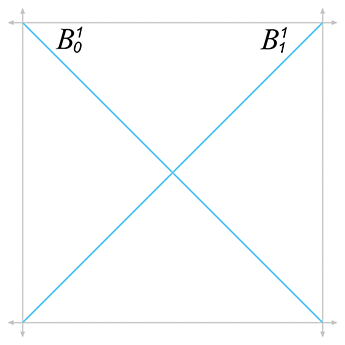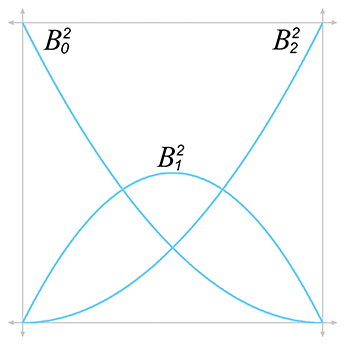${B}_{0}^{1}\left(t\right)=1-t$ ${B}_{0}^{2}\left(t\right)=\left(1-t{\right)}^{2}$ ${B}_{1}^{1}\left(t\right)=t$ ${B}_{1}^{2}\left(t\right)=2\left(1-t\right)t$ ${B}_{2}^{2}\left(t\right)={t}^{2}$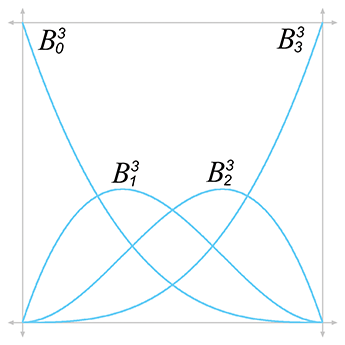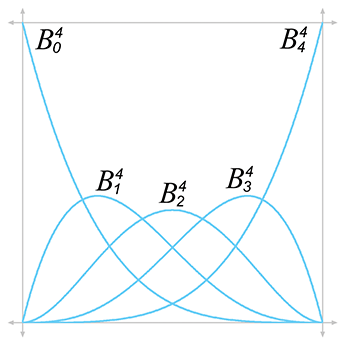${B}_{0}^{3}\left(t\right)=\left(1-t{\right)}^{3}$ ${B}_{0}^{4}\left(t\right)=\left(1-t{\right)}^{4}$ ${B}_{1}^{3}\left(t\right)=3t\left(1-t{\right)}^{2}$ ${B}_{1}^{4}\left(t\right)=4t\left(1-t{\right)}^{3}$ ${B}_{2}^{3}\left(t\right)=3{t}^{2}\left(1-t\right)$ ${B}_{2}^{4}\left(t\right)=6{t}^{2}\left(1-t{\right)}^{2}$ ${B}_{3}^{3}\left(t\right)={t}^{3}$ ${B}_{3}^{4}\left(t\right)=4{t}^{3}\left(1-t\right)$ ${B}_{4}^{4}\left(t\right)={t}^{4}$
Figure 13.13Bernstein polynomials of degrees 1–4

The function ${B}_{i}^{n}\phantom{\rule{negativethinmathspace}{0ex}}\left(t\right)$ is a Bernstein polynomial, named after Sergei Bernstein (1880–1968).16 We've already figured out the pattern of these polynomials, but here's the precise formula:

Bernstein polynomial

Figure 13.13 shows the graphs for the Bernstein polynomials up to the quartic case.

The properties of the Bernstein polynomials tell us a lot about how Bézier curves behave. Let's discuss a few properties in particular.

Sum to one. The Bernstein polynomials sum to unity for all values of $t$ , which is nice because if they didn't, then they wouldn't define proper barycentric coordinates. This fact is not immediately obvious, neither from visual inspection of Figure 13.13 nor from a cursory examination of the equations, but it can be proven. If you relish the idea of working through such a proof for the quadratic case, check out Exercise 4.

Convex hull property. The range of the Bernstein polynomials is $0\dots 1$ for the entire length of the curve, $0\le t\le 1$ . Combined with the previous property, this means that Bézier curves obey the convex hull property: the curve is bounded to stay within the convex hull of the control points. Compare this with the Lagrange basis polynomials, which do not stay within the $\left[0,1\right]$ interval, causing polynomial interpolation to not obey the convex hull property. One manifestation of this is the undesirable “overshooting” witnessed in Figure 13.4.

Endpoints interpolated. The first and last polynomials attain unity when we need them to. Because ${B}_{0}^{n}\phantom{\rule{negativethinmathspace}{0ex}}\left(0\right)=1$ and ${B}_{n}^{n}\phantom{\rule{negativethinmathspace}{0ex}}\left(1\right)=1$ , the curve touches the endpoints. Notice that $t=0$ and $t=1$ are the only places where any of the basis polynomials reach 1, which is why the other control points are only approximated and not interpolated.

Global support. All the polynomials are nonzero on the open interval $\left(0,1$ )—that is, the entire curve excluding the endpoints. The region where the blending weight for a control point is nonzero is called the support of the control point. Wherever the control point has support, it exerts some influence on the curve.

Bézier control points have global support because the Bernstein polynomials are nonzero everywhere other than the endpoints. The practical result is that when any one control point is moved, the entire curve is affected. This is not a desirable property for curve design. Once we have a section of the curve that looks how we want, we would prefer that editing of some other distant control point not disturb the section that was shaped the way we liked it. This envious situation, known as local support, occurs when we move a particular control point and only the part of the curve near that control point is affected, for some definition of “near.”

Local support means that the basis function is nonzero only in some interval, and outside this interval it is zero. Unfortunately, such a basis function cannot be described as a polynomial, and thus no polynomial curve can achieve local control. However, local support is possible by piecing together small curves that fit together just right to form a spline, as Section 13.6 discusses.

One local maximum. Although each control point exercises influence over the entire curve, each exerts the most influence at one particular point along the curve. Each Bernstein polynomial ${B}_{i}^{n}\phantom{\rule{negativethinmathspace}{0ex}}\left(t\right)$ , which serves as the blend weight for the control point ${\mathbf{b}}_{i}$ , has one maximum at the auspicious time $t=i/n$ . Furthermore, at that time, ${\mathbf{b}}_{i}$ exerts more weight than any other control point.

Thus, although every point on the interior of the curve is influenced to some degree by all the control points (because Bézier control points have global support), the nearest control point has the most influence.

## 13.4.3Bézier Derivatives and Their Relationshipto the Hermite Form

Let's take a look at the derivatives of a Bézier curve. Since we like to use the cubic curve as our example, we're talking about the velocity and acceleration of the curve. Remember that the velocity is related to the tangent (direction) of the curve, and the acceleration is related to its curvature.

Section 13.1.6 showed how to get the velocity function of a curve from the monomial coefficients:

Position and velocity of a cubic curve